# SurfStat

## A Matlab toolbox for the statistical analysis of univariate and multivariate surface and volumetric data using linear mixed effects models and random field theory

Developed by Keith J. Worsley (1951-2009)Orginally hosted on http://www.math.mcgill.ca/keith

Moved here to ensure continuity.

SurfStat is a Matlab toolbox for the statistical analysis of univariate and multivariate surface and volumetric data using linear mixed effects models and random field theory. It is inspired by Jason Lerch's Thickness Statistics written in R, and Jonathan Taylor's BrainStat, part of NIPY, written in Python. It is intended for cortical thickness data on triangular meshes, either for the whole cortex or one for each hemisphere. It will handle any triangulated surface data, written in FreeSurfer or MNI object format. The only requirement is that the triangulation scheme must be the same for all surfaces, i.e. the data must be registered to a common surface.

### Reference:

Worsley, K.J., Taylor, J.E., Carbonell, F., Chung, M.K., Duerden, E., Bernhardt, B., Lyttelton, O., Boucher, M., Evans, A.C. (2009). SurfStat: A Matlab toolbox for the statistical analysis of univariate and multivariate surface and volumetric data using linear mixed effects models and random field theory. NeuroImage, OHBM poster, accepted.

### Features of the legacy version (21 February 2009)

• SurfStat now does statistical analysis for volumetric data, e.g. VBM, DBM and PET data.
• New viewers added for volumetric data.
• Memory mapping has been added to cope with very large data sets. There is now essentially no limit on the size of the data, but you will need some free disk space, equal to twice the size of the data, for scratch space.
• Its main engine fits fixed effects and mixed effects, univariate and multivariate, linear models and makes inference using T, F, Hotelling's T2 and Roy's maximum root statistics.
• Random field theory (RFT) for peaks and clusters, as well as False Discovery Rate (FDR).
• Model formula rather than a design matrix for specifying the linear model.
• Mixed effects models fitted by ReML.
• Off-the-shelf Matlab graphics that are ready to publish.
• FAST! everything loaded into memory.
• Truly interactive analysis, no need for batch.
• A mere 200K of code ...

Thanks to Oliver Lyttelton for suggesting the multivariate statistics, Moo Chung and Cate Hartley for help with FreeSurfer, Boris Bernhardt for helping with the mixed effects, and Emma Duerden for constant feedback that has really improved the package.

### User applications

• Moo Chung has used SurfStat for Amygdala Surface Modeling (see the last section).
• Boris Bernhardt has used SurfStat for cortical thickness in temporal lobe epilepsy (see the end of his web page).

### Contents

• Installation
• Documentation and help
• Viewing the data
• Reading the cortical thickness data
• Principal Components Analysis
• Model Formula
• Fitting the model
• Main effect for gender
• Main effect for age
• Interaction
• F statistics
• Connectivity
• ROI analysis
• Volume to surface and vice-versa
• Mixed effects, a.k.a. longitudinal data, a.k.a. repeated measures
• Memory mapping
• Smoothing
• Multivariate data statistical analysis
• VBM data
• DBM data
• PET data
• PET data: more elaborate linear models
• Future features
• References for random field theory

### Installation

• Unzip the contents of surfstat.zip into a directory called e.g. `C:\Program Files\MATLAB\R2006a\toolbox\local/surfstat/`.
• Go to the Matlab window and click File --> Set Path... ---> Add Folder ... ---> `C:\Program Files\MATLAB\R2006a\toolbox\local/surfstat/` --> OK --> Save.

### Documentation and help

Here is documentation for all the functions in SurfStat, thanks to Guillaume Flandin's m2html. If you want your own copy of this documentation, download it here, unzip the file, and point your web browser to the `doc/SurfStat` directory.

You can get complete on-line documentation about any Matlab function, including the ones in SurfStat, by typing `help` followed by the name of the function, e.g.

help colormap help SurfStatReadSurf help term or you can email me - I love to get feed-back!

### Viewing the data

There is a FreeSurfer example at the end of this section, but throughout the rest of the web page, we shall analyse 147 subjects from the ICBM data base. The data is stored in MNI object files. The scientific questions are not very exciting, but I have chosen them just to illustrate the ideas:
• Is cortical thickness related to gender?
• Is cortical thickness related to age?
• Does the age effect depend on gender?
• Does cortical thickness show any "functional" connectivity, and does the connectivity depend on age or gender?

First let's read in one subject whose id is 100:

s100 = SurfStatReadSurf( {... 'c:/keith/fMRI/ICBM/surfaces/mni_icbm_00100_mid_surface_left_81920.obj',... 'c:/keith/fMRI/ICBM/surfaces/mni_icbm_00100_mid_surface_right_81920.obj'} ); t100 = SurfStatReadData( {... 'c:/keith/fMRI/ICBM/thickness/mni_icbm_00100_native_rms_rsl_tlink_20mm_left.txt',... 'c:/keith/fMRI/ICBM/thickness/mni_icbm_00100_native_rms_rsl_tlink_20mm_right.txt'} ); The first thing you will want to do is to look at the data: SurfStatView( t100, s100, 'Cort Thick (mm), Subj 00100' );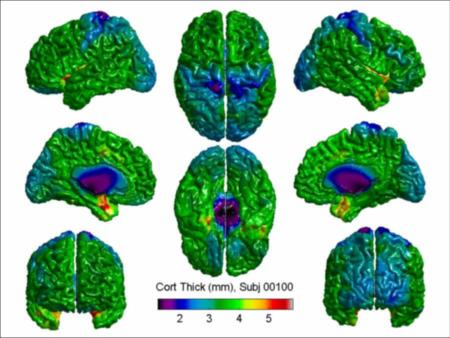and perhaps save this as a .jpg file for publication or PowerPoint:

saveas( gcf, 'c:/keith/surfstat/figs/subject100.jpg' ) You can make the lettering smaller by increasing the paper size before saving (maybe there is an easier way!). I have set it to 6 × 4.5, but to make the lettering smaller, try the Matlab default of 8 × 6: set(gcf,'PaperPosition',[0.25 2.5 8 6]) saveas( gcf, 'c:/keith/surfstat/figs/subject100.jpg' ) You can play with figures quite a bit using the tool bar in the Matlab figure window. For example, you can click the "Rotate 3D" button, then rotate the images separately, or you can click the "Zoom In" button. The "Data Cursor" button allows you to click on a point and pull off the x,y,z coordinates, the index number (id) of the point, and its value.

The point is marked by the little black and white square in the top centre figure. Its id is 66180, so you can type

>> t100(66180) 2.5400 to get the value, or to get the x,y,z coordinates: >> s100.coord(:,66180) 31.6937 -49.7099 74.2872 Some people prefer a black background: SurfStatView( t100, s100, 'Cort Thick (mm), Subj 00100', 'black' ); If you are unhappy with the colour map, then try one of the Matlab alternatives: SurfStatColormap( 'jet' ); A common adjustment is the limits on the colour bar, for which there is a special function: SurfStatColLim( [3 4] );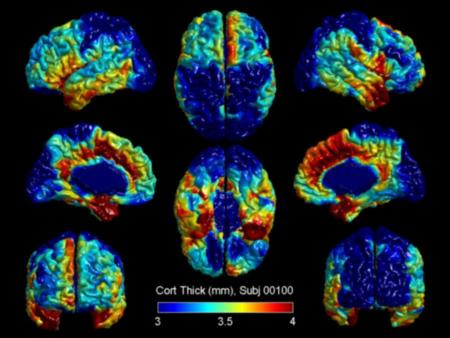For further customizing, see the code of the `SurfStatViewData.m` function.

You may just want to work with one hemisphere:

s100left = SurfStatReadSurf( ... 'c:/keith/fMRI/ICBM/surfaces/mni_icbm_00100_mid_surface_left_81920.obj'); t100left = SurfStatReadData( ... 'c:/keith/fMRI/ICBM/thickness/mni_icbm_00100_native_rms_rsl_tlink_20mm_left.txt' ); SurfStatView( t100left, s100left, 'Cort Thick (mm), Subj 00100' );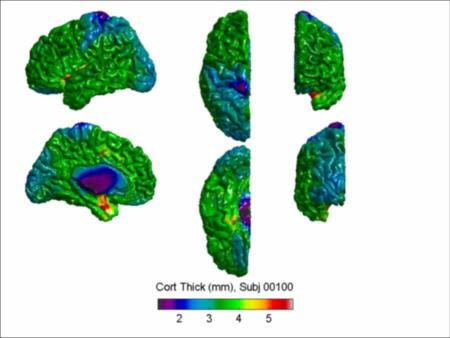Finally a FreeSurfer example, courtesy Moo Chung:

s = SurfStatReadSurf( {... 'c:/keith/surfstat/FreeSurferdata/lh.pial', ... 'c:/keith/surfstat/FreeSurferdata/rh.pial'} ); t = SurfStatReadData( {... 'c:/keith/surfstat/FreeSurferdata/lh.thickness', ... 'c:/keith/surfstat/FreeSurferdata/rh.thickness'} ); SurfStatView( t, s, 'Cort Thick (mm), FreeSurfer data' );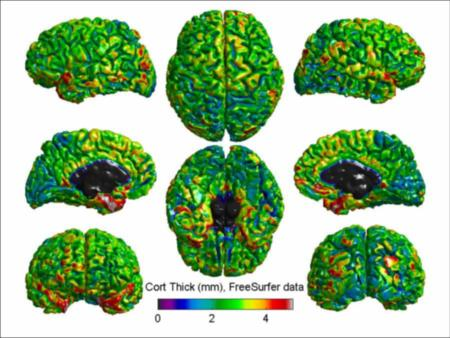You can check that the FreeSurfer surface has no topological deformities by calculating its Euler characteristic (EC):

SurfStatResels(s) 4 The answer is 4, which is what you would get for a pair of spherical surfaces. However there are other non-spherical surfaces with the same EC, so you can only say that if the EC were not 4, then it would not be a pair of spherical surfaces.

First you have to make a list of all the names of the files. I have excluded 5 of the 152 subjects because their data was bad or incomplete, and written `SurfStatListDir.m` to list the contents of a directory: excludefiles = [ '00220'; '00338'; '00111'; '00128'; '00137' ]; filesleft = SurfStatListDir( 'c:/keith/fMRI/ICBM/surfaces/mni_icbm_00*_mid_surface_left_81920.obj', excludefiles ); filesright = SurfStatListDir( 'c:/keith/fMRI/ICBM/surfaces/mni_icbm_00*_mid_surface_right_81920.obj', excludefiles ); filesboth = [ filesleft, filesright ]; The only reason you need all the surfaces is to find the average surface: avsurf = SurfStatAvSurf( filesboth ); SurfStatWriteSurf( 'c:/keith/fmri/icbm/av.obj', avsurf ); This takes 2 minutes on my 2-year-old laptop, so instead we shall read it in directly: avsurf = SurfStatReadSurf( 'c:/keith/fmri/icbm/av.obj' ); Now we need a mask to exclude the inter-hemispheric cut: mask = SurfStatMaskCut( avsurf ); SurfStatView( mask, avsurf, 'Masked average surface' );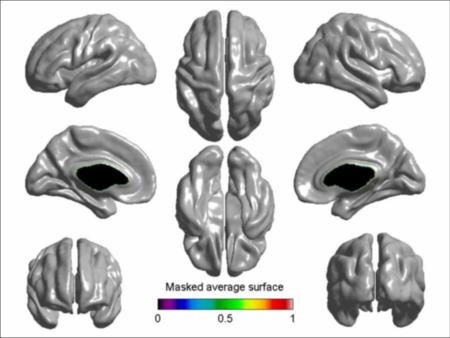You might want to also exclude the brain stem. To do this, I clicked on where I thought the centre of the brain stem was: (0; -16; -8)mm, and I clicked a bit further out to guess the radius: 20mm. Then you can add a ball-shaped ROI to the mask as follows (see ROI):

mask = mask & SurfStatROI( [0; -16; -8], 20, avsurf ) == 0; SurfStatView( maskb, avsurf, 'Masked average surface -brainstem' );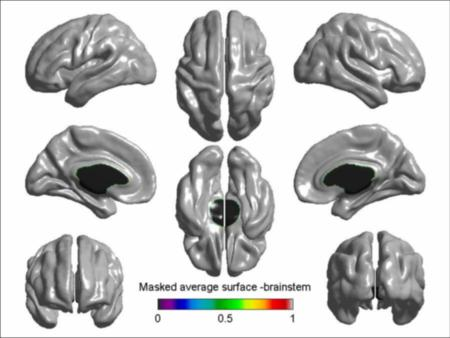Since I don't really know where the brain stem is, I shall just stick to the first mask in what follows.

### Reading the cortical thickness data

Let's suppose the file names and covariates are in a file similar to what we use for `glim_image`, i.e. a text file with fields for the thickness file names, and the covariates: sub_1_left.txt subj_1_right.txt female 19.6906 sub_2_left.txt subj_2_right.txt female 24.8378 sub_3_left.txt subj_3_right.txt male 29.8617 sub_4_left.txt subj_4_right.txt male 22.7214 sub_5_left.txt subj_5_right.txt male 34.8118 ... This can be read by the standard Matlab `textread`: [thickfileleft, thickfileright, gender, age] ... = textread( 'c:/keith/fMRI/ICBM/glim.txt', '%s %s %s %f' ); clf; hist( age ); xlabel('age');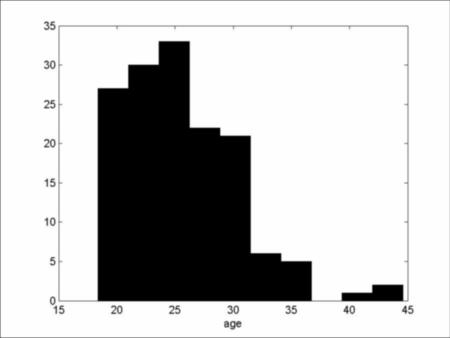To read in the actual thickness data from the above files:

Y=SurfStatReadData( [thickfileleft, thickfileright] ); To save memory, this is stored as single precision (4 bytes), but all subsequent calculations (except smoothing) are done in double precision (8 bytes).

Let's take a look at the mean thickness for vertices:

meanthick = mean( double( Y ) ); SurfStatView( meanthick, avsurf, 'Mean thickness (mm), n=147' );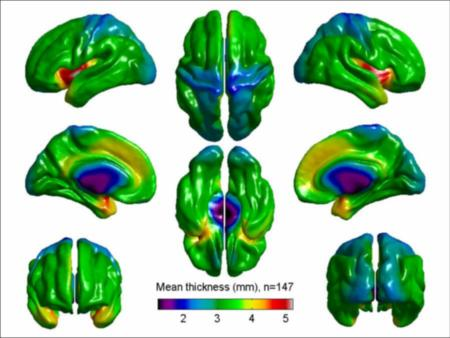We can save this for later:

save c:/keith/surfstat/data/meanthick meanthick Let's take a look at the mean thickness for subjects: meanthicksubj = mean( double( Y(:, mask) ), 2 ); SurfStatPlot( age, meanthicksubj );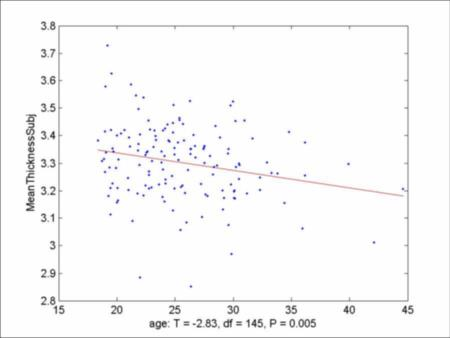As expected, thickness decreases with age. The line looks a bit faint, and the markers are too small. You can change the line width and marker size as follows:

SurfStatPlot( age, meanthicksubj, [], [], 'LineWidth',2, 'MarkerSize',12 );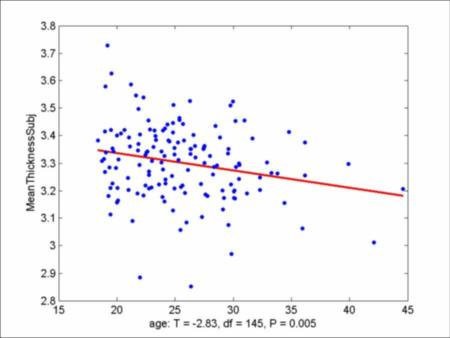or you can edit the figure by clicking on the "Edit Plot" button (the one with the little arrow) in the Figure toolbar, then double-clicking on the feature you want to edit.

For gender:

SurfStatPlot( gender, meanthicksubj );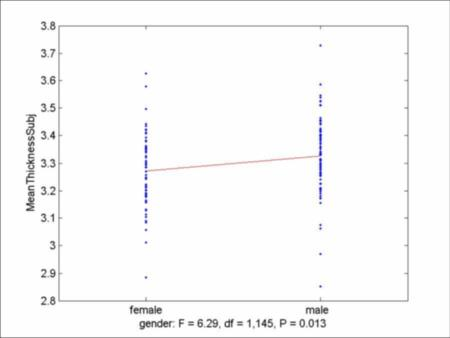Males appear to have overall thicker cortex than females.

FreeSurfer group registered data is often stored in two `.mgh` files. In the following example, data on 18 subjects is in two files. To read these in:

Y = SurfStatReadData( { 'c:/keith/surfstat/FS/mgh/lh.thickness.mgh',... 'c:/keith/surfstat/FS/mgh/rh.thickness.mgh' } ); `Y` is an 18 × 327684 matrix of cortical thickness data on the 18 subjects. To read in one of the surfaces: s = SurfStatReadSurf( { 'c:/keith/surfstat/FS/mgh/lh.pial',... 'c:/keith/surfstat/FS/mgh/rh.pial' } ); and view the average thickness: SurfStatView( mean( Y ), s, 'Mean of 18 registered FS thicknesses');

### Principal Components Analysis

Principal Components Analysis is a useful exploratory tool for checking the data or discovering connectivity. [ pcntvar, U, V ] = SurfStatPCA( Y, mask ); pcntvar 33.5008 4.0641 3.3371 2.3098 The first component is by far the largest, explaining 33.5% of the variability of the data. SurfStatView( V(1,:), avsurf, 'First Principal Component, 33.5% variance' );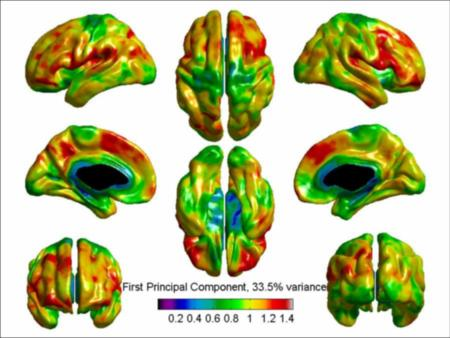It looks as if this is a "whole brain" component, i.e. the thickness on the entire surface is positively correlated. An explanation is that some subjects have overall thick cortex, some have overall thin cortex, perhaps due to differences in contrast at the grey-white boundary. See `SurfStatNorm.m` to normalize the cortical surface data, either by subtracting or dividing the global mean. However before doing this, let's plot the subject component against age:

SurfStatPlot( age, U(:,1) ); title('First Principal Component, 33.5% variance');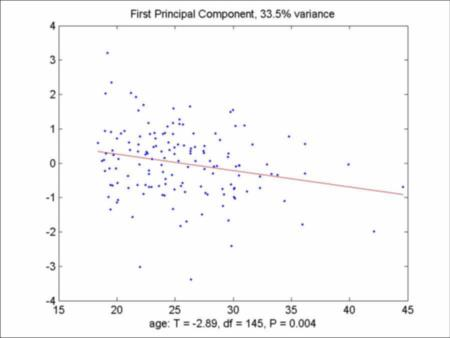The global effect decreases with age; in other words, it could be just an age-related atrophy effect. This makes sense: since the spatial component is roughly constant across the brain, then the subject component must be the global average, which we have already noted decreases with age.

Now let's look at the second component, which explains a mere 4.1% of the variability:

SurfStatView( V(2,:), avsurf, 'Second Principal Component, 4.1% variance' );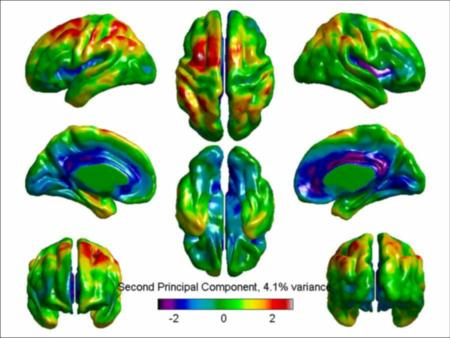Plotting the subject component against gender:

SurfStatPlot( gender, U(:,2) ); title('Second Principal Component, 4.1% variance');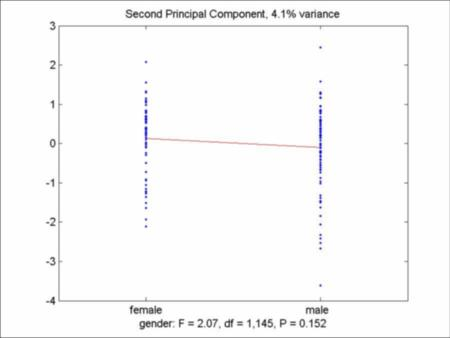We note that the second component might be a gender effect. Females score positively on this component, so they have higher cortical thickness in the red areas, lower cortical thickness in the blue areas, compared to males.

Higher order components seem to be more difficult to interpret.

### Model Formula

This is the fun bit: specifying the model by a model formula, rather than a design matrix. Model formulas were pioneered by GenStat and GLIM in the early 1970's, and are now used in most statistics packages such as R and SAS. To show you the idea, let's cut it down to the first 5 subjects: >> age5 = age(1:5) 19.6906 24.8378 29.8617 22.7214 34.8118 >> gender5 = gender(1:5) 'female' 'female' 'male' 'male' 'male' The idea is to convert variables into a newly defined Matlab class called a `term`. For the technically minded, a `term` has two components: a matrix, and a cell of strings for the names of the columns. The function `term.m` converts a vector or a cell array of strings to a `term`, which is displayed as follows: >> Age = term( age5 ) age5 --------- 19.6906 24.8378 29.8617 22.7214 34.8118 >> Gender = term( gender5 ) female male -------------- 1 0 1 0 0 1 0 1 0 1 `double` and `char` convert a `term` back to its matrix and name components. The operators `+`, `*`, `^` and `-` have been redefined (overloaded) for `term`s so that they match the operation of terms in a model formula. You now write down a model formula (without the coefficients) e.g. a main effect of Age and Gender: >> 1 + Age + Gender 1 age5 female male -------------------------- 1 19.6906 1 0 1 24.8378 1 0 1 29.8617 0 1 1 22.7214 0 1 1 34.8118 0 1 Perhaps you suspect that the age effect depends on gender, i.e. you want to add an interaction between Age and Gender; >> 1 + Age + Gender + Age*Gender 1 age5 female male age5*female age5*male --------------------------------------------------------- 1 19.6906 1 0 19.6906 0 1 24.8378 1 0 24.8378 0 1 29.8617 0 1 0 29.8617 1 22.7214 0 1 0 22.7214 1 34.8118 0 1 0 34.8118 This could also be written as >> (1 + Age)*(1 + Gender) 1 age5 female age5*female male age5*male --------------------------------------------------------- 1 19.6906 1 19.6906 0 0 1 24.8378 1 24.8378 0 0 1 29.8617 0 0 1 29.8617 1 22.7214 0 0 1 22.7214 1 34.8118 0 0 1 34.8118 Perhaps a quadratic effect of Age? >> 1 + Age + Age^2 1 age5 age5*age5 ------------------------------ 1 19.6906 387.71973 1 24.8378 616.91631 1 29.8617 891.72113 1 22.7214 516.26202 1 34.8118 1211.8614 or a cubic Age effect? >> (1 + Age)^3 1 age5 age5*age5 age5*age5*age5 ---------------------------------------------- 1 19.6906 387.71973 7634.43408 1 24.8378 616.91631 15322.8439 1 29.8617 891.72113 26628.3088 1 22.7214 516.26202 11730.1958 1 34.8118 1211.8614 42187.0774 Maybe you don't want a term, such as the constant term >> (1 + Age)^3 - 1 age5 age5*age5 age5*age5*age5 ------------------------------------ 19.6906 387.71973 7634.43408 24.8378 616.91631 15322.8439 29.8617 891.72113 26628.3088 22.7214 516.26202 11730.1958 34.8118 1211.8614 42187.0774

### Fitting the linear model

Back to the full data, let's specify a main effect of age and gender: Age = term( age ); Gender = term( gender ); M = 1 + Age + Gender 1 age female male --------------------------------- 1 19.6906 1 0 1 24.8378 1 0 1 29.8617 0 1 1 22.7214 0 1 1 34.8118 0 1 ... You can see this as an SPM-style image: image( M );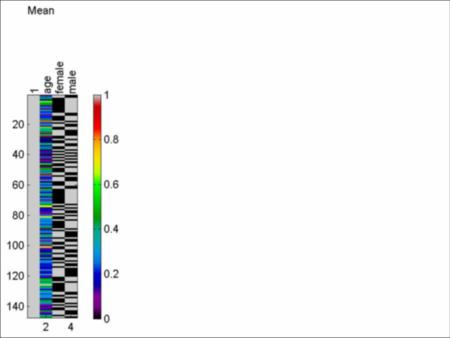(The empty space on the right is reserved for the design matrix for the variance of a mixed effects model.) Now let's fit the linear model, saving the results in `slm`:

slm = SurfStatLinMod( Y, M, avsurf ); In case you are interested, you can see what's in `slm` by typing >> slm X: [147x4 double] df: 144 coef: [4x81924 double] SSE: [1x81924 double] tri: [163840x3 int32] resl: [245760x1 double] `slm.X` is the design matrix, `slm.df` is the error degrees of freedom, `slm.coef` is the coefficients, `slm.SSE` is error sum of squares, `slm.tri` is the list of triangle indices and `slm.resl` is a function of the resels along each edge. Normally you never need to look at any of these things!

### Main effect for gender

Let's look at the T statistic for male-female. To set up the contrast, we specify it in terms of the observations rather than in terms of the coefficients (see `help SurfStatT` for more information on this): contrast = Gender.male - Gender.female -1 -1 1 1 1 ... `contrast` is a numeric 147 × 1 vector. Note how `Gender.male` gives you the numeric indicator variable for `male`, i.e. 1 if the observation is `male`, and 0 otherwise. Then to get the T statistic: slm = SurfStatT( slm, contrast ) X: [147x4 double] df: 144 coef: [4x81924 double] SSE: [1x81924 double] tri: [163840x3 int32] resl: [245760x1 double] c: [7.9797e-017 1.3010e-018 -1.0000 1.0000] k: 1 ef: [1x81924 double] sd: [1x81924 double] t: [1x81924 double] The contrast in the columns of the design matrix X is in `slm.c`. It equals [0 0 -1 1] (to machine accuracy), i.e. column 4 - column 3. `slm.k` is the number of variates (univariate in this case). Effects are in `slm.ef`, and their standard deviations (standard errors) are in `slm.sd`. The T statistic is in `slm.t`, and by multiplying it by `mask`, we set all the values outside the mask to zero, which makes it better to look at: SurfStatView( slm.t.*mask, avsurf, 'T (144 df) for males-females removing age' );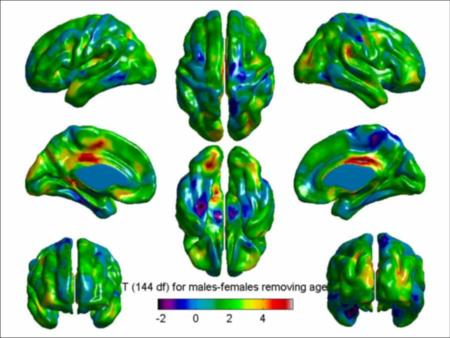To find the threshold for P=0.05, corrected, the resels are:

resels = SurfStatResels( slm, mask ) 2.0000 19.6153 578.5045 stat_threshold( resels, sum(mask), 1, slm.df ) 4.4259 However the best way is to view the P-values for each vertex. [ pval, peak, clus ] = SurfStatP( slm, mask ); `pval.P` contains P-values for peaks, and `pval.C` contains P-values for clusters. This special structure is recognised by `SurfStatView` which draws the figure in a special way: SurfStatView( pval, avsurf, 'Males-females removing age' );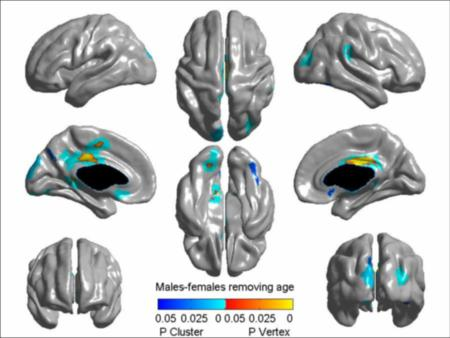Maybe there is something hidden; try inflating the average brain:

avsurfinfl = SurfStatInflate( avsurf ); SurfStatView( pval, avsurfinfl, 'Males-females removing age' );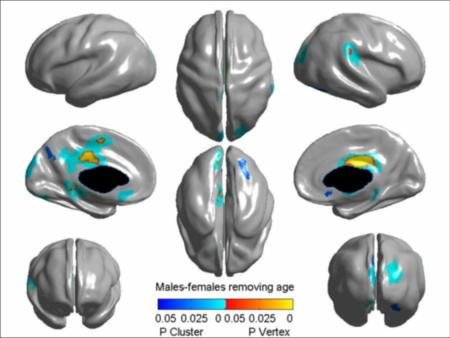If you want to inflate it more, try

avsurfinfl = SurfStatInflate( avsurf, 0.75 ); `peak` and `clus` contain a list of peaks and clusters, together with their P-values, as in FmriStat. They can be displayed nicely by converting them to a `term`: >> term( clus ) clusid nverts resels P ---------------------------------------- 1 710 8.7714 2.5675e-007 2 1460 6.4006 2.5699e-007 3 546 5.6912 2.5865e-007 ... 12 187 0.95521 0.033553 13 37 0.93294 0.036895 14 102 0.81846 0.060139 15 161 0.53477 0.19819 ... There are 13 clusters whose extent is significant at P=0.05. The peaks are: >> term( peak ) t vertid clusid P ------------------------------------ 5.7658 70593 1 0.0002103 5.7541 70586 1 0.00022173 5.7433 70557 1 0.00023325 ... 4.4435 29130 2 0.046866 4.4326 75010 4 0.048814 4.419 39444 5 0.051243 4.4067 39760 3 0.053529 ... Where is the largest peak with id=70593? Here are the x,y,z coordinates in mm on the average surface: >> avsurf.coord(:,70593) 5.9320 -23.6405 29.8528 If you want all the coordinates in mm added to the table, try this: >> term( peak ) + term( SurfStatInd2Coord( peak.vertid, avsurf )', {'x','y','z'}) t vertid clusid P x y z -------------------------------------------------------------------- 5.7658 70593 1 0.0002103 5.93198 -23.6405 29.8528 5.7541 70586 1 0.00022173 5.52406 -19.2547 30.3012 5.7433 70557 1 0.00023325 4.73798 -12.3197 30.9665 ... 4.4435 29130 2 0.046866 -1.34981 -8.22836 27.8238 4.4326 75010 4 0.048814 30.8152 -77.5804 25.1885 4.419 39444 5 0.051243 -16.2635 -82.7056 -12.8587 4.4067 39760 3 0.05353 -20.5747 -36.3622 1.15415 ... How does the data look at the largest peak? Here's a plot against gender, adjusting for age: SurfStatPlot( gender, Y( :, 70593 ), age );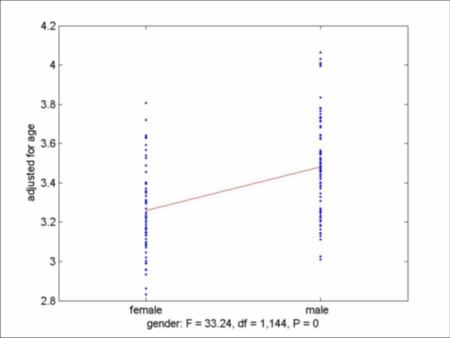Note that the the F statistic on the plot is the square of the maximum T statistic for the contrast, i.e. 33.24 = 5.7658^2. F statistics with one degree of freedom always equal the square of T statistics. Moreover the P-values are also the same, i.e. the P-value of the F is the P-value of the 2-sided T (equal to twice the P-value of the one-sided T).

Finally, Q values or False Discovery Rate:

qval = SurfStatQ( slm, mask ); SurfStatView( qval, avsurf, 'Males-females removing age' );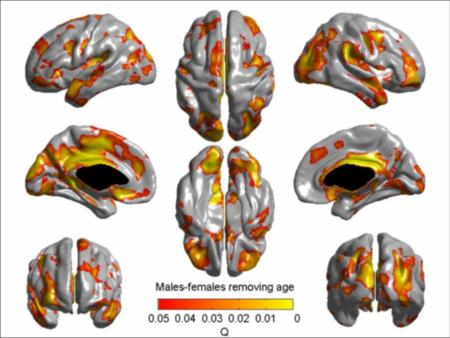Recall that:

• RFT controls the probability of ever finding a false positive - you will never report a false positive, 19 times out of 20.
• FDR controls the proportion of false positives amongst your discoveries - you will always report false positives, but not too many (1/20).

• RFT is useful for confirmation (e.g. scientific publication) - you want to be sure that all your discoveries are real.
• FDR is useful for exploration (e.g. drug discovery: costs are in \$\$\$) - you want to make discoveries, but not too many bad ones.

### Main effect for age

Now try T statistics for a negative effect of age on cortical thickness: slm = SurfStatT( slm, -age ); SurfStatView( slm.t.*mask, avsurf, 'T (144 df) for -age removing gender' );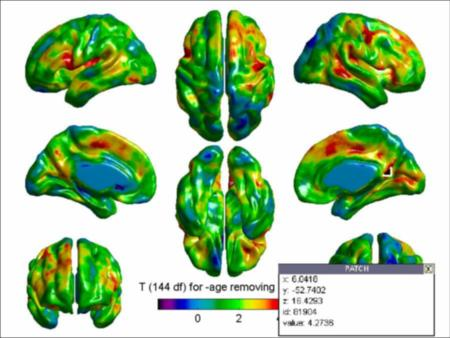Let's try to click on the maximum T statistic with the "Data Cursor" button. I can't hit it exactly - the cursor is just to the right of the mask in the middle right figure. To find the id try:

>> find( slm.t == max( slm.t ) ) 81887 To plot the cortical thickness at this point against age, adjusting for gender: Yseed = double( Y(:,81887) ); SurfStatPlot( age, Yseed, gender );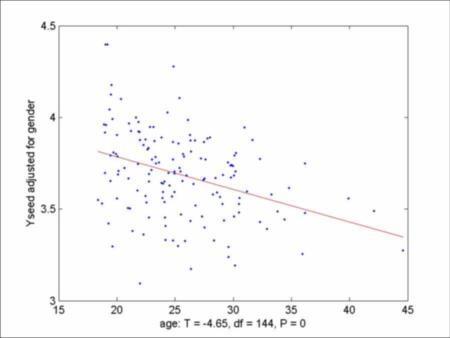P and Q-values, in one line:

SurfStatView( SurfStatP( slm, mask ), avsurf, '-Age removing gender' );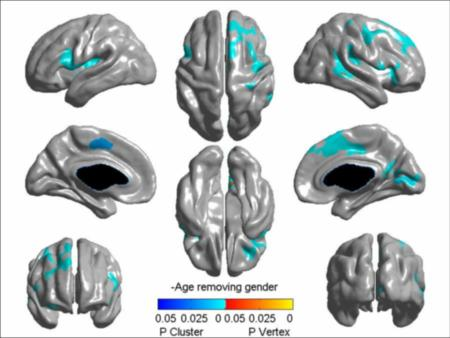Only clusters are significant, and not peaks. This suggests that the age effect covers large regions, rather than local foci. This agrees with our conclusion above that there is age-related atrophy right across the brain. FDR tells the same story:

SurfStatView( SurfStatQ( slm, mask ), avsurf, '-Age removing gender' );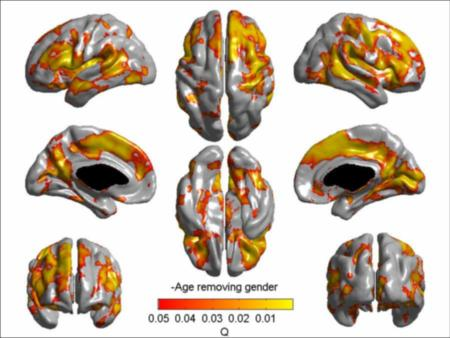### Interaction

Is the age effect the same for males and females? Test for an interaction between Age and Gender: slm = SurfStatLinMod( Y, 1 + Age + Gender + Age*Gender, avsurf ); slm = SurfStatT( slm, age.*Gender.male - age.*Gender.female ); SurfStatView( slm.t.*mask, avsurf, 'T (143 df) for age*(male-female)' );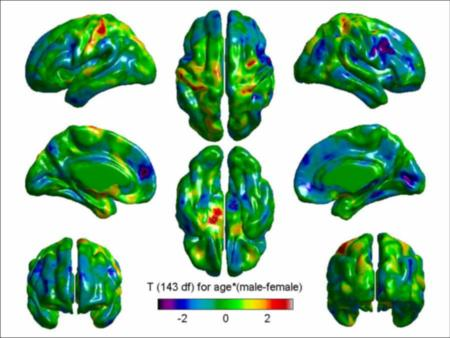Nothing doing here. Nevertheless, let's take a look at what is happening at the seed point. Here's a plot of `Yseed` against `age` with two separate lines for each `gender`:

SurfStatPlot( age, Yseed, 1, gender );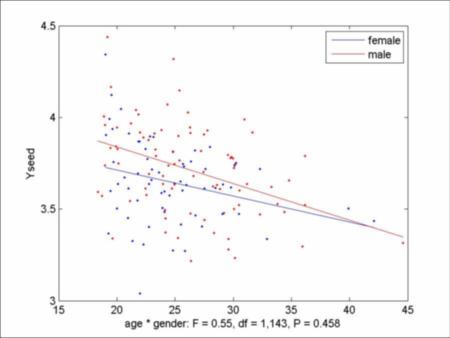As before, a negative effect of age (atrophy), but little difference in slope between the two genders.

### F statistics

F statistics are obtained by comparing nested models. For example, let's compare the above model with interaction to the null model with just a constant, which can be fitted without bothering with the surface in the third argument: slm0 = SurfStatLinMod( Y, 1 ); slm = SurfStatF( slm, slm0 ); SurfStatView( slm.t.*mask, avsurf, 'F statistic, 3,143 df' ); F statistics are in `slm.t`, and their degrees of freedom are in `slm.df`=[3 143].

The P and Q values are calculated using the same functions. The software knows that `slm.t` is an F statistic, rather than a T statistic, because an F statistic has two degrees of freedom:

SurfStatView( SurfStatP( slm, mask ), avsurf, 'F statistic, 3,143 df' );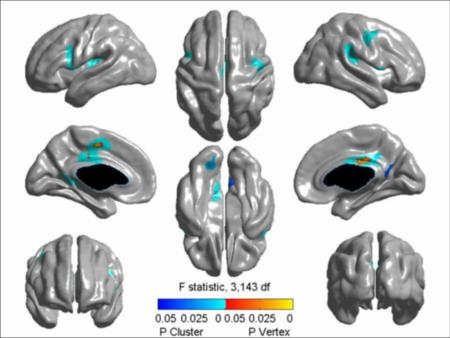SurfStatView( SurfStatQ( slm, mask ), avsurf, 'F statistic, 3,143 df' );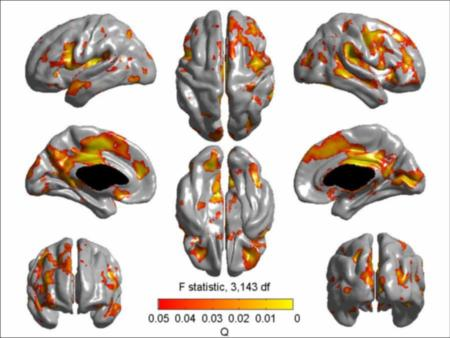### Connectivity

Let's take the seed as the peak of the age effect above. It is located just to the right of the mask in the middle right figure. Is the cortical thickness at this point correlated with the rest of the cortical surface, allowing for an effect of age and gender? slm = SurfStatLinMod( Y, 1 + Age + Gender + term(Yseed), avsurf ); slm = SurfStatT( slm, Yseed ); SurfStatView( slm.t.*mask, avsurf, 'Connectivity' ); SurfStatColLim( [0 5] ); Of course the T statistic is infinite at the seed itself, so we needed to reset the colour limits:

A large part of the brain is correlated with the seed. However I would claim that pure connectivity as above is of little scientific interest. A more interesting question is how the connectivity changes with say gender. To answer this, add an interaction between seed and the rest of the model, then look at the interaction between seed and gender:

slm = SurfStatLinMod( Y, ( 1 + Age + Gender )*( 1 + term(Yseed) ), avsurf ); slm = SurfStatT( slm, Yseed.*Gender.female - Yseed.*Gender.male ); SurfStatView( slm.t.*mask, avsurf, 'Female connectivity - male connectivity' );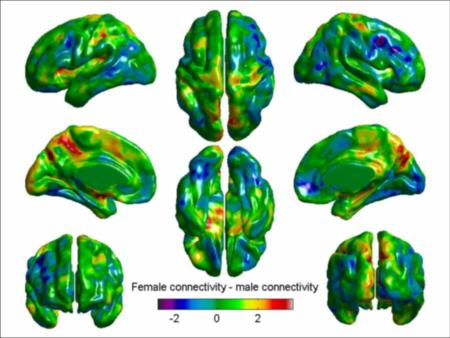There is really no evidence for a gender effect on connectivity. Nevertheless, let's take a look at the most significant peak at `id=3698` tucked inside the left temproal lobe. The T statistic is `slm.t(3698)=3.7024`. To see how the connectivity differs between genders, here's a plot of the data `Y3698` at that point. The data is adjusted for `Age` and its interaction with `Yseed`, and plotted against `Yseed` for each gender:

Y3698 = Y( :, 3698 ); M = ( 1 + Age )*( 1 + term(Yseed) ); SurfStatPlot( Yseed, Y3698, M, gender);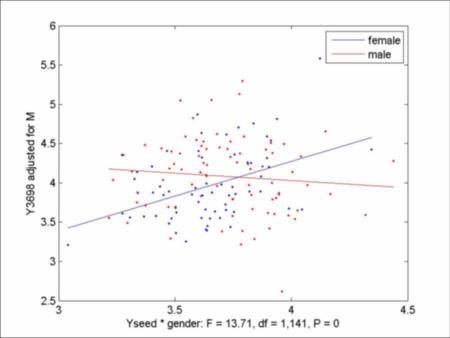As you can see, the connectivity is more in females than males, which is why the T statistic was positive. The F statistic is `13.71=3.7024^2` as expected.

How does the connectivity change with age?

slm = SurfStatT( slm, Yseed.*age ); SurfStatView( slm.t.*mask, avsurf, 'Age effect on connectivity' ); SurfStatColLim( [-5 5] ); Again there were some spurious large values in the vicinity of the seed, so we had to reset the colour limits:

This looks more promising; for confirmation:

SurfStatView( SurfStatP( slm, mask ), avsurf, 'Age effect on connectivity' );This time there is one small significant cluster where connectivity increases with age ... or maybe this is just the 1 time out of 20 when we expect to see a false positive!

Unfortunately there is no easy way to make a plot that show the effect of an interaction between two continuous variables (here `Yseed` and `age`).

### ROI analysis

If you have a surface atlas handy, you can read in a mask for an ROI just like thickness data, but taking the values 1 inside the ROI and 0 outside. If you have a volumetric atlas handy, see below. If you don't have an atlas handy, you can make a circular ROI centred at a point, as follows. You specify the centre either as `[x; y; z]` coordinates or by a vertex id. For example, one of our users was interested in a region in the top right figure. We clicked on the centre and found the `id`=53815. To make the ROI with a radius of 10mm: id = 53815; maskROI = SurfStatROI( id, 10, avsurf );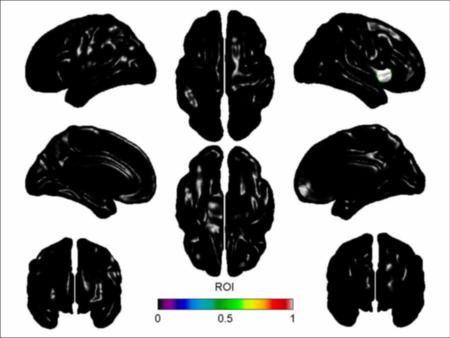The region shows up as white in the top right figure, just behind the temporal lobe.

You could also make an ROI from a cluster. Let's take the clusters of the T statistic for age in the model `M = Age + Gender`. The variable `clusid` contains the cluster id's for each vertex, obtained by adding a fourth output argument to `SurfStatP`:

slm = SurfStatT( SurfStatLinMod( Y, 1 + Age + Gender, avsurf ), -age ); [ pval, peak, clus, clusid ] = SurfStatP( slm, mask ); SurfStatView( pval, avsurf );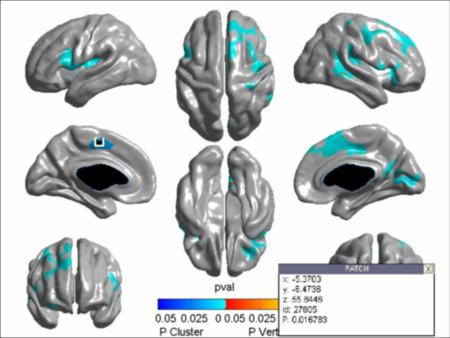(The Figure is the same as before.) Suppose we want to make an ROI from the dark blue cluster. First click on any point in the cluster, find its `id`=27805 (its P-value is 0.016783), then

clusid( 27805 ) 10 is its cluster id number (10). We can now make an ROI out of cluster 10: maskROI = clusid == clusid( 27805 ); By adding a third argument to `SurfStatP` (see `help SurfStatP`) you can change the threshold for defining clusters to any arbitrary value; by default it is the uncorrected P=0.001 threshold.

You can find clusters for any (non-statistical) image using `SurfStatPeakClus`, as follows. Suppose we want to form an ROI from the mean thickness, as here. First make a structure, say `s`, with two fields `t` containing the data, and `tri` containing the triangles (`s` is arbitrary, but you must use `t` and `tri` as fields):

s.t = mean(Y); s.tri = avsurf.tri; [ peak, clus, clusid ] = SurfStatPeakClus( s, mask, 4 ); SurfStatView( clusid, avsurf, 'Mean thickness (mm) > 4' );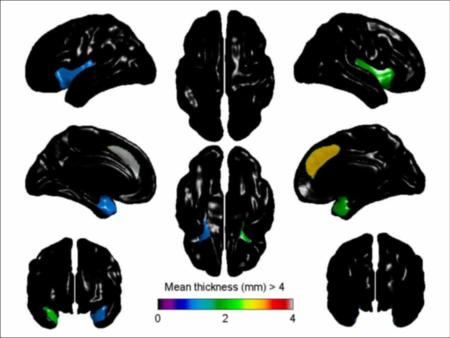The figure shows the cluster id's (by coincidence there are four clusters). To make an ROI out of the green cluster (`clusid`=2, read from the colour bar in the figure):

maskROI = clusid == 2; ROI's can be combined using the Matlab logical operations. Suppose you had two ROI's `maskROI1` and `maskROI2`. Using the Matlab "or" operator `|`, their union is maskROI = maskROI1 | maskROI2; To get their intersection, use the "and" operator `&`.

Going back to the linear model with age and gender, we can find the resels of the first ROI:

slm = SurfStatLinMod( Y, 1 + Age + Gender, avsurf ); reselsROI = SurfStatResels( slm, maskROI ) 1.0000 5.7081 4.6337 Note that the first component is 1, which is the Euler Characteristic, suggesting that our ROI is a single connected region and does not contain any fragments or holes. If we wanted to search for peaks within the ROI (not likely since it is so small!) we should use a T statistic P=0.05 threshold of 3.0254: stat_threshold( reselsROI, sum(maskROI), 1, slm.df) 3.0254 Usually we want to average the data within the ROI and analyze that: YROI = mean( Y(:, maskROI), 2 ); This will give you a vector of the average thickness inside the ROI. To plot it against age, adjusting for gender: SurfStatPlot( age, YROI, gender );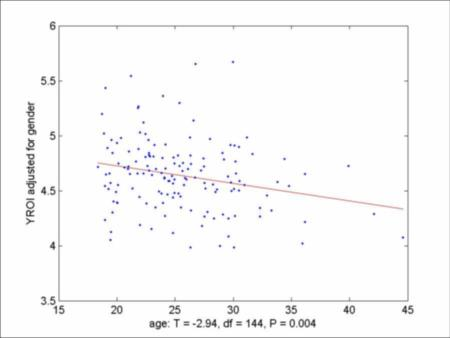`YROI` can be treated exactly like `Y` itself, but without specifying the surface, e.g.

slmROI = SurfStatLinMod( YROI, 1 + Age + Gender ); slmROI = SurfStatT( slmROI, -age ) X: [147x4 double] df: 144 coef: [4x1 double] SSE: 14.3026 ef: 0.0159 sd: 0.0054 t: 2.9428 Obviously FDR is irrelevant, but to get P-values use SurfStatP( slmROI ) P: 0.0019 You could also get F statistics in the usual way: slmROI = SurfStatF( slmROI, slm0ROI ) SurfStatP( slmROI )

### Volume to surface and vice-versa

Most brain imaging data, such as atlases, are volumetric, so we need a handy way to get volumetric data onto surfaces. To do this we interpolate the volume onto the surface of each subject, then average. Unfortunately reading and writing volume data is messy, because it comes in so many different formats. Readers and writers are provided for MINC (`.mnc`), ANALYZE (`.img`), NIFTI (`.nii`) or AFNI (`.brik`) format. All the matlab functions for ANALYZE (`.img`) and NIFTI (`.nii`) are included with SurfStat. If you are using AFNI (`.brik`) formatted files, you will need to install the AFNI Matlab Library and set the matlab path as here.

If you are using MINC (`.mnc`) formatted files you will need the extra tools in the emma toolbox also available for Windows and Linux. Installing the Windows and Linux versions is a bit tricky. In the zipped file that you download, there are `.dll` files and `.exe` files - these must be moved to a directory in the operating system path, e.g. the `matlab/bin/win32` directory. Also you must create a directory `c:/tmp` or `/tmp` for emma to use as scratch space. Finally you must set the matlab path to the emma directory, as here. To test it out, see if you can read the MINC template file supplied with SurfStat:

fid = fopen( 'icbm_template_2.00mm.mnc' ) template = fopen( fid ) fclose( fid ) vol = SurfStatReadVol1( template ) A common error at this stage is forgetting to move the `.dll` files and `.exe` files into the path of the operating system. If you don't get any red error messages, it has worked! Now check to see if you can write a MINC file: vol.file_name = 'test.mnc'; SurfStatWriteVol1( vol ) A common error at this stage is forgetting to make the `c:/tmp` or `/tmp` directories. Again if there are no red warning messages, you should have written a MINC file called `test.mnc` in your working directory (it will just contain zeros). Congratulations!

Now we are ready for the real thing. First read in your volumetric data from `c:/keith/surfstat/myfile.mnc`:

vol = SurfStatReadVol1( 'c:/keith/surfstat/myfile.mnc' ); Once that is done, we then read in all the surface data (this takes a few minutes): surfs = SurfStatReadSurf( filesboth ); To interpolate the volume to the surface: s = SurfStatVol2Surf( vol, surfs ); SurfStatView( s, avsurf ); To do the reverse, and interpolate the surface data in `s` to a volume `vol2`, then write it to `c:/keith/surfstat/myfile2.mnc`: vol2 = SurfStatSurf2Vol( s, surf); vol2.file_name = 'c:/keith/surfstat/myfile2.mnc'; SurfStatWriteVol1( vol2 ); It is instructive to view the two files `c:/keith/surfstat/myfile.mnc` and `c:/keith/surfstat/myfile2.mnc` side by side. If you are using MINC files, then `register` is convenient (go here for a Windows version). You can call it up from within Matlab as follows: !"C:\Program Files\MATLAB\R2007b\toolbox\local\emma\register.exe" -rgb c:/keith/surfstat/myfile.mnc c:/keith/surfstat/myfile2.mnc & You will see that `c:/keith/surfstat/myfile2.mnc` has chunks of data missing in places where there are no cortical surfaces. Also you will notice that `c:/keith/surfstat/myfile2.mnc` is a lot smoother looking than the original `c:/keith/surfstat/myfile.mnc` because the surfaces that it interpolates onto are all different. The different surfaces have the effect of blurring the data.

### Mixed effects, a.k.a. longitudinal data, a.k.a. repeated measures

Mixed effects models are used when the observations are not independent with equal variances. This happens when there is longitudinal data or repeated measurements on a subject, which tend to be positively correlated, or when there are different variances for different groups of subjects. Both can be handled by mixed effects models. The simplest case is when the data set consists of subjects each with several measurements. The data set just analysed only has one measurement per subject, so there is no need for a mixed effects analysis. So to illustrate the methods, I shall create a fake data set. I shall pretend that all subjects with the same age (in years) and gender are the same "Subject" (never do this in real life!). The result is 35 "Subjects" with indices as follows (I have sorted the data by age within gender, so that the output looks nicer): [ u, i, subj ] = unique( [ floor( age ) Gender.male ], 'rows' ); num2str(subj') 2 2 2 2 2 2 2 2 4 4 4 4 4 4 6 6 6 6 6 6 6 8 8 8 8 8 8 8 10 10 10 10 10 12 12 12 12 12 12 14 14 14 14 14 14 14 16 16 16 18 18 18 20 20 22 22 22 24 24 24 27 27 33 34 1 1 1 1 1 3 3 3 3 3 5 5 5 7 7 7 7 7 9 9 9 9 9 11 11 11 11 11 11 13 13 13 13 13 13 13 13 13 13 15 15 15 15 17 17 17 17 17 17 19 19 19 19 19 21 21 21 21 23 23 23 23 23 23 23 25 25 25 25 25 25 25 26 26 28 29 29 30 30 31 32 32 35 Subj = term( var2fac( subj ) ); The last line converts `subj` to a cell array of strings, then to a term. What can we do with `Subj`? There are three choices; the first one is to ignore `Subj`, as above: M1 = 1 + Age + Gender; image( M1 );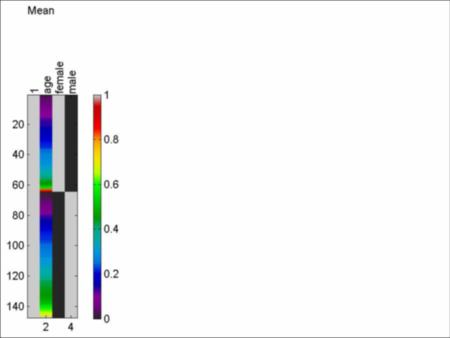Suppose in fact there are correlations between observations on the same subject. Then coefficients of fixed effects (`Age` and `Gender`) are still unbiased but less precise. But most importantly, their estimated standard deviations are biased (usually too small), so that the resulting T statistics are wrong (usually too big). One possibiility is to allow for `Subj` as a fixed effect:

M2= 1 + Age + Gender + Subj; image( M2 );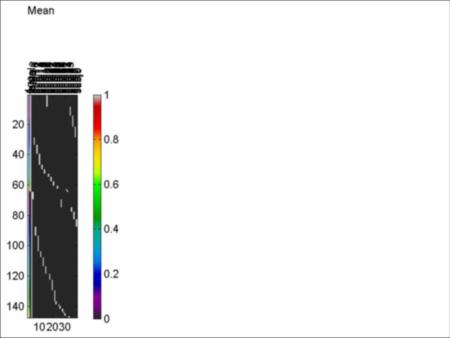(The image is a horrible mess because the 35 `Subj` indicators dominate.) This model also gives unbiased estimates of fixed effects (`Age` and `Gender`), but again they are less precise. The reason is that by allowing for a fixed effect of `Subj`, all our inference comes from differences within a subject. We are throwing away information from age or gender differences between subjects. In particular, we are throwing away any subject with just one observation, i.e. the 5 subjects (#28, 31, 33, 34, 35). We are only using age information within a subject. It is as if all subjects had the same age (in years).

Age effects are only inferred from the monthly effect within each subject, and information about age effects between subjects is lost. Nevertheless, inference about the age effects that remain are valid even if the observations within a subject are equally correlated. In other words, the estimated standard deviation of the age effects are unbiased, and T statistics have the correct null distribution. This analysis is better than `M1`, at least for age.

However it is a disaster for gender: it is now impossible to estimate gender, because gender only varies between subjects, and we have just removed a subject effect!.

The solution is to try a mixed effects model:

M3 = 1 + Age + Gender + random( Subj ); image( M3 );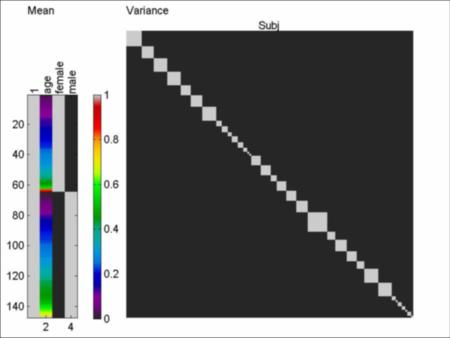Here we have made `Subj` into a random effect, meaning that its coefficients are independent Gaussian random variables. This induces equal correlations between observations on the same subject. On the right you will see the model for the variance of the observations. We have forgotten to add the identity matrix `I` to allow for independent "white" noise in every observation (this is added by default to any fixed effect model, but it must be specifically added to a mixed effects model):

M3 = 1 + Age + Gender + random( Subj ) + I; image( M3 );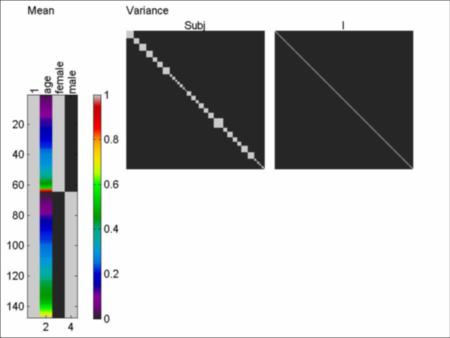The variance is now a linear model with two "variables", a matrix with 1's in each subject, plus the identity matrix with 1's down the diagonal. This mixed effects model `M3` lies somewhere between the two fixed effects models `M1` and `M2`. In `M3`, if the variance of `random( Subj )` is 0, you get `M1`; if the variance of `random( Subj )` is infinite, you get `M2`. Model `M3` estimates a variance in between. You might be curious to know that if the design is balanced then inference about the age effect is the same in `M2` as in `M3`. A balanced design has the same number of observations at the same time instants (e.g. at ages 21.0, 21.5, 21.75 years) on each subject.

The rest of the analysis proceeds as before. `SurfStatLinMod` fits model `M3` by first fitting `M1`. It then uses the residuals to estimate the variance model by ReML. The fixed effects and the overall variance are re-estimated, again by ReML. Thus all parameter estimates are approximately unbiased. If this procedure were iterated, it would converge to the ReML estimates, but this takes much more time, and the resulting estimates are not much different. If you are worried about this, you should use Jason Lerch's Thickness Statistics which calls R's `nlme`, but it is much more time consuming (up to 1 day per fit!). `nlme` will also fit more elaborate models such as an AR(p) time-series model for the correlation structure, which is not implemented in SurfStat.

Here we go:

slm = SurfStatLinMod( Y, M3, avsurf ) SurfStatView( slm.r.*mask, avsurf, 'Correlation within subject' );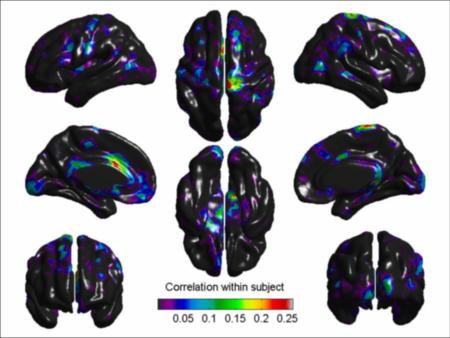`slm.r` contains the estimated correlation within subjects. It is clamped to a minimum of a small positive number to avoid a non-positive definite variance matrix. Most of the image is in fact clamped, indicating no correlations within "subjects". This is what we expect, since the "subjects" are fake. The results for a negative age effect (atrophy) are:

slm = SurfStatT( slm, -age ) mean( slm.dfs( find(mask) ) ) 54.9440 SurfStatView( slm.t.*mask, avsurf, 'T stat for -age, 54.9 df' );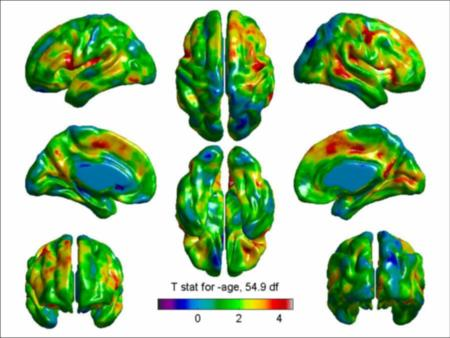The first thing to note is that the degrees of freedom is not equal to #observations - #variables = 147 - 3 = 144 as for `M1`. It is not even an integer. It is a non-integer effective degrees of freedom that varies spatially (since the variance model varies spatially), and is available in `slm.dfs`. Its average inside the mask is 54.9, and this is used for the P-value calculations (not shown). For a gender effect:

slm = SurfStatT( slm, Gender.male - Gender.female ) mean( slm.dfs( find(mask) ) ) 25.3734 SurfStatView( slm.t.*mask, avsurf, 'T stat for male-female, 25.4 df' );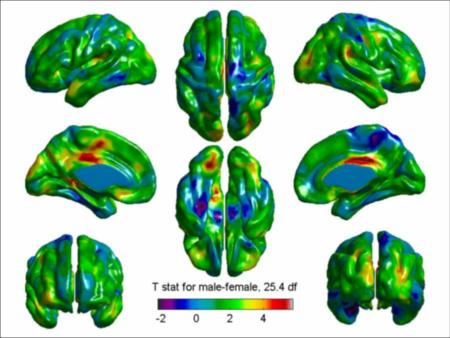Note that you get different degrees of freedom for different contrasts, unlike for `M1`. Now the degrees of freedom is less. Why? Essentially because gender is estimated between subjects, and there are only 35 subjects, so the degrees of freedom has to be less than 35.

More elaborate models are possible. It is common practice to assume that the interaction between a fixed effect and a random effect is also random. In the case of age, this allows for a random age slope for different subjects. The test for the age main effect still makes sense: it is testing to see if the mean of these random slopes is different from 0. To do this:

M = 1 + Age + Gender + random(Subj) + Age*random(Subj) + I; image( M );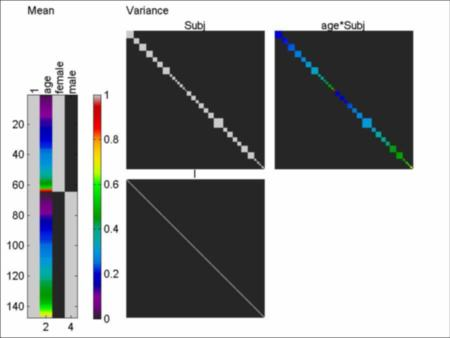It turns out that this model is not appropriate because it is not invariant to a rescaling of age. If for instance we measured age from a baseline of (say) 18 years, then the fitted model would be different. To overcome this, we need to allow for a covariance between the random age-subject interaction and the random subject main effect, which can be written in two identical ways:

M = 1 + Age + Gender + ( 1 + Age )*random(Subj) + I; M = Gender + ( 1 + Age )*( 1 + random(Subj) ) + I; image( M );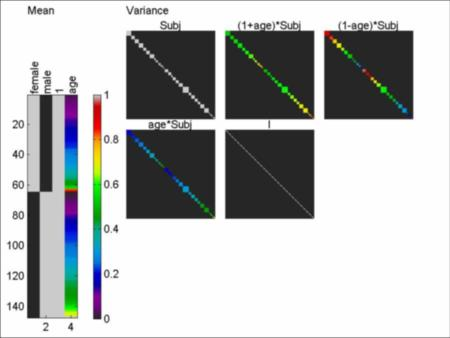Now there are two extra components that allow for the covariance. As a result, fitting takes a little longer:

slm = SurfStatT( slm, -age ) mean( slm.dfs( find(mask) ) ) 12.6669 SurfStatView( slm.t.*mask, avsurf, 'T stat for -age, 12.7 df' );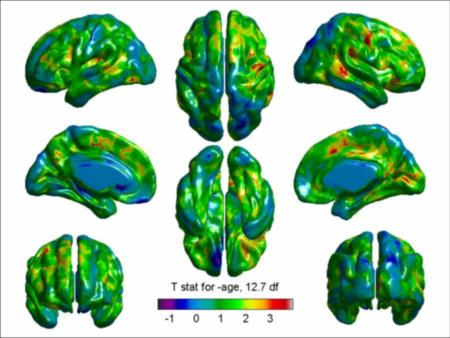The df has gone down from 54.9 to 12.7. The reason is that if we assume that there is a different age slope for each subject, then the age sd is now estimated between the 30 subjects with at least two observations, so the age sd has to have less than 30 degrees of freedom. The 30 is reduced even more, since more weight is put on the few subjects with larger numbers of observations. In general, you lose degrees of freedom as the variance model becomes more elaborate, which means you lose sensitivity to detect effects.

So my advice is to stick to simple models, such as `M3`, unless there is strong evidence to the contrary. You might be curious to know that FmriStat (and all the other common packages such as SPM and FSL) do in fact allow for an interaction between fixed effects and random effects. This is implemented by finding one slope per subject, throwing the rest of the data away, then combining the slopes in another linear model. The reason why it is appropriate for fMRI data is that the number of repeated measures in an fMRI time series is huge (100-200) compared to the number of repeated measures here (1-10). This means that sd's can be estimated very accurately within subjects, there is no need to pool sd information between subjects, and so no need for a complex mixed effects model.

Finally, it is possible to do Welch's T test, which tests for a difference in group means allowing for a difference in variance. For example, to test for a difference in gender, allowing for different variances for males and females, but ignoring age and subject,

M = 1 + Gender + Gender * I; image( M );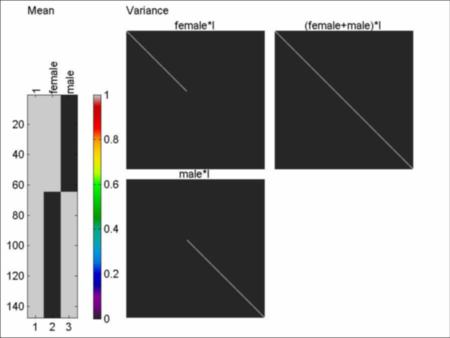Actually the model is over-parameterized. To reduce the model by eliminating redundant variables both for mean and variance:

M = redmod ( M ); image( M );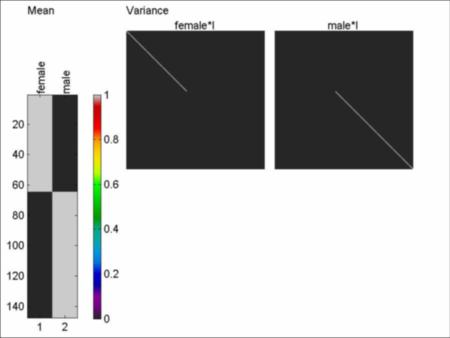Now it is clearer what is going on: there is a separate mean for males and females, and a separate variance for males and females. The effective degrees of freedom is 137.3, down slightly from the 147 - 2 = 145 without allowing for separate variances, which is the price you pay for allowing for separate variances.

The most general model would be to interact all fixed effects with all random effects:

M = redmod( ( 1 + Age + Gender )*( 1 + random( Subj ) ) + I ); image( M );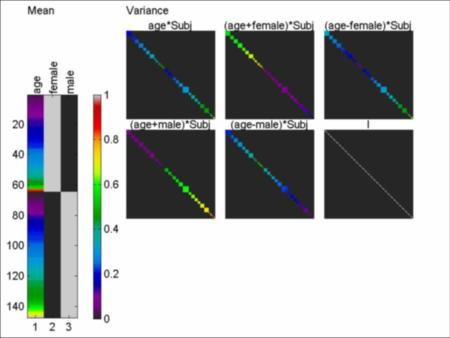Apart from taking a long time to fit, such a model leaves too little degrees of freedom to make any useful inference.

So far only T tests for univariate mixed effects models are implemented - F tests and multivariate mixed effects models are yet to come.

A final note of caution: the methods used to fit mixed effects models are only approximate. Convergence is not guaranteed. The way in which the model is forced to have a positive definite variance matrix restricts the types of correlation structures. The method is not completely invariant to a linear re-parameterization, even if fixed effects terms are added before crossing with a random effect as recommended above. However the algorithm will give exact results for a single random effect if the design is balanced. It will also give exact results for Welch's test even if the grouping factor has more than one group. In general it will give good results whenever there is just one additive random effect, such as `random(Subj)`, so my suggestion is to stick to that.

### Memory mapping

This section can be skipped unless you are curious about what happens "under the hood". For most purposes, you will not need to know what memory mapping is, or how it works, or even when it is being used, because all `SurfStat` functions handle memory mapping without you knowing. However there might be occasions when you need to know where your data is really stored, in which case, read on.

When the amount of data is big, it can exceed the available memory. In this case, the three functions that read data (`SurfStatReadSurf`, `SurfStatReadData`, `SurfStatReadVol`) all resort to memory mapping instead of reading the data into memory. Memory mapping is invoked if the total storage exceeds 64Mb of memory. You can change this memory limit to e.g. 128MB by issuing the following command:

global MAXMEM; MAXMEM=128; or by adding an extra argument - see the `help` for the reader.

Memory mapping works as follows. The data is first written to a temporary file as 4 byte reals. The temporary file is in a directory `SurfStat` in the same directory as the first file to be read in. This directory will be created if it doesn't exist already. If it can't be created because you don't have permission, then an error message will be produced prompting you to supply a directory where you do have permission to write. Note that neither the temporary file, nor the temporary directory, are ever deleted. To avoid wasted disk space, you should delete these files after you have quit Matlab.

Then the output parameter of the reader, say `Y`, is a special structure that contains information on the name of the file where the data is stored, the format, and the dimensions of the data array (see below for an example). You can access the data by:

Y.Data(1).Data If the data is 2D, then this is a an array of dimensions (#vertices × #data files). If the data is 3D, then this is a an array of dimensions (#vertices × #components or coordinates × #data files). For example, to access 2D data at vertex 1000, files 5 to 20, use: Y.Data(1).Data( 1000, 5:20 ) To access 3D data at vertices 100, 200, 400, all components, and file 6 use: Y.Data(1).Data( [100 200 400], :, 6 ) You can treat this exactly like an ordinary variable; you can for instance copy the data to a variable: Y1 = Y.Data(1).Data( 1000, 5:20 ) or write a variable to the memory mapped data: Y.Data(1).Data( [100 200 400], :, 6 ) = Y2 All the `SurfStat` functions will handle memory mapped data where appropriate.

As an example of memory mapping, we shall analyze the trivariate coordinates of the surfaces themselves. We shall see if coordinates depend on age allowing for a difference in coordinates between males and females. First we must read in all the surface data (this takes a few minutes):

surfs = SurfStatReadSurf( filesboth ); Since the amount of data 147 × 81924 × 3 × 4 = 138Mb exceeds the limit of 64Mb, then the data is memory mapped rather than read into memory. In the case of `SurfStatReadSurf`, only `surfs.coord` is memory mapped, and `surfs.tri` is read into memory in the usual way. To see this, type: >> surfs tri: [163840x3 int32] coord: [1x1 memmapfile] Investigating further: >> surfs.coord Filename: 'c:\keith\fMRI\ICBM\surfaces\SurfStat\tp95705ccb_c494_43e5_991a_fed16698f279' Writable: true Offset: 0 Format: {'single' [81924 3 147] 'Data'} Repeat: Inf Data: 1x1 struct array with fields: Data `Filename` is the memory mapped file. It is mapped as a 81924 × 3 × 147 single precision array. To access all the coordinates of vertex 1000 on subjects 20 and 25: >> surfs.coord.Data(1).Data( 1000, :, [20 25] ) -2.8642 16.7308 -4.1040 -0.6023 19.9366 -4.6526 To view the surface of subject 15: test.coord = surfs.coord.Data(1).Data( :, :, 15 )'; test.tri = surfs.tri; SurfStatView( [], test, 'Subj 1000' );

### Smoothing

To better detect differences over a broader region, we shall first smooth the coordinates read in above. How much to smooth? It depends on how big the features are that you wish to detect. To detect 20mm features, you should use a 20mm FWHM filter. Of course you never know how big the features are in advance, so you have to make a guess. Since the cortical thickness data was smoothed 20mm, we shall do the same to the coordinates: Y = SurfStatSmooth( surfs.coord, avsurf, 10 ); The last parameter, 10, is the FWHM in mesh units. Since the mesh size of our data is about 2mm on average, then this will give roughly 20mm smoothing. Note that smoothing is usually the most time-consuming step; the above smoothing took 26 minutes on my 2-year-old laptop! To take a look at the smoothed coordinates of the first subject (00100), coloured by cortical thickness (compare this to the unsmoothed coordinates above): s100smooth.coord = Y.Data(1).Data( :, :, 1 )'; s100smooth.tri = surfs.tri; SurfStatView( t100, s100smooth, 'Subj 00100 smoothed 20mm' );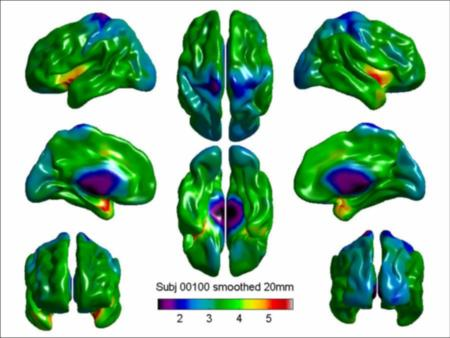### Multivariate data statistical analysis

Statistical analysis now proceeds exactly as for univariate data, except that the T statistic is now the maximum over all linear combinations of the variates. In other words, we take the maximum of the T statistics for the x coordinate, the y coordinate, the z coordinate, and all possible linear combinations such as x+y or 0.1*x+0.4*y-0.3*z. This maximum T is known as Hotelling's T (the square root of Hotelling's T2). The same idea applies to the F statistic, which is now the maximum F over all linear combinations. This is known as Roy's maximum root. Random field corrected P-values for peaks and clusters are available.

As an example, let's look for an age effect on the smoothed surface coordinates, allowing for gender.

slm = SurfStatLinMod( Y, Gender + Age, avsurf ); slm = SurfStatT( slm, age ); h = SurfStatView( slm.t.*mask, avsurf, 'Max T for age effect on coordinates' );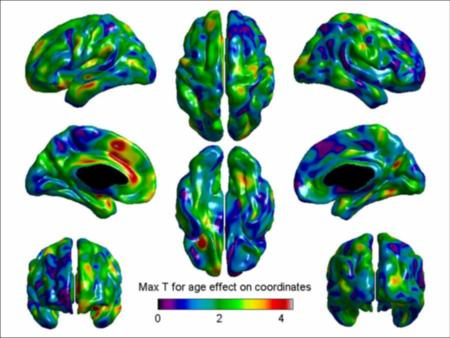It's interesting to compare this with the T statistic for an age effect on cortical thickness. However before getting excited, we should find out the corrected threshold:

resels = SurfStatResels( slm, mask ) 2.0000 4.5114 228.9485 stat_threshold( resels, length(slm.t), 1, slm.df, [], [], [], [], slm.k ) 5.1849 Note that this is larger than the 4.4259 for the univariate T-statistic (even though the resels are smaller) - this is the price you pay for searching over all linear combinations of the multivariate data to get the maximum T statistic.

Unfortunately the P-values for peaks and clusters are not significant:

[ pval, peak, clus ] = SurfStatP( slm, mask ); term( peak ) t vertid clusid P --------------------------------- 4.3592 1806 1 0.83991 4.2794 28210 2 1.0707 4.1982 16539 3 1.3633 term(clus) clusid nverts resels P ------------------------------------- 1 53 0.016794 0.30263 2 14 0.0041732 0.5022 3 6 0.00024013 0.68522 Why is `P>1` for the last two peaks? The reason is that `P` approximates the expected number of peaks above `t`, which only approximates the P-value if `P` is small, say less than 0.1. Both the P-value and Q-value images are blank, so we don't show them, but here's the code: SurfStatView( pval, avsurf, 'Max T for age effect on coordinates' ); SurfStatView( SurfStatQ( slm, mask ), avsurf, 'Max T for age effect on coordinates' ); Even though the peak at 1806 is not significant, here are the age effects on the x, y and z coordinates, their sd, and (univariate) T statistics: [ slm.ef(:, 1806) slm.sd(:, 1806) slm.ef(:, 1806)./slm.sd(:, 1806) ] 0.0528 0.0237 2.2242 0.0434 0.0306 1.4179 0.0742 0.0223 3.3248 Note that the univariate T statistics are all less than the maximum T statistic of 4.3586 (obviously). If this was significant, it would suggest, for example, that z coordinates increase by 0.07 ± 0.02 mm per year at this point near the right cingulate.

Here's an even better way of showing the movement of the surface with age. We can use Matlab's `quiver3` to show the age effects in `slm.ef` as little arrows. First let's pick three local maxima in the table above as origins for the arrows, then find their coordinates (the rows are `x`, `y`, `z`, the columns are the vertices):

id = [ 1806 28210 16539 ]; coord = SurfStatInd2Coord( id, avsurf ) -9.2008 -6.5057 -5.4116 16.7131 14.8676 36.2732 33.1974 50.9881 14.2673 Now for a bit of Matlab code. We want to add the arrows to each of the axes in the above figure. Luckily we have saved the axis handles in `h` by putting `h` as the output of the `SurfStatView` function above. Then we loop over axes, adding red arrows in the positive direction and blue arrows in the negative direction, so we can always see the arrows: for i = 1:8 axes( h(i) ); hold on; quiver3( coord( 1, : ), coord( 2, : ), coord( 3, : ), ... slm.ef( 1, id ), slm.ef( 2, id ), slm.ef( 3, id ), ... 'LineWidth',2, 'Color','red' ); quiver3( coord( 1, : ), coord( 2, : ), coord( 3, : ), ... -slm.ef( 1, id ), -slm.ef( 2, id ), -slm.ef( 3, id ), ... 'LineWidth',2, 'Color','blue' ); hold off; end Let's zoom in on one of the axes by hitting the "+" magnifying glass in the figure tool bar:

(Note that the arrows are not to scale.) The red arrows are pointing up and away from the brain centre, suggesting a movement of anatomy in that direction with increasing age. However we shouldn't read too much into this, because the P-values are not significant.

Finally, let's test for a quadratic age effect, allowing for gender, using the maximum F statistic (Roy's maximum root):

slm0 = SurfStatLinMod( Y, Gender ); slm = SurfStatLinMod( Y, Gender + Age + Age^2, avsurf ) slm = SurfStatF( slm, slm0 ); SurfStatView( slm.t.*mask, avsurf, 'Max F for quadratic age effect (2,143 df)' );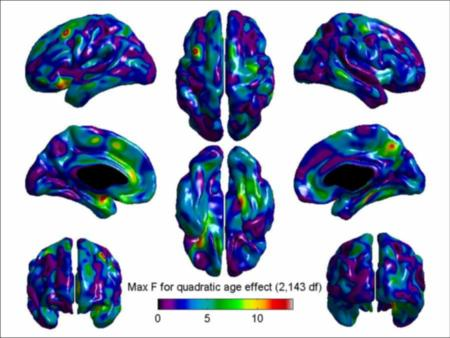The corrected P-values are not significant, but there is one significant cluster:

[ pval, peak, clus ] = SurfStatP( slm, mask ); term( peak ) t vertid clusid P ---------------------------------- 13.5506 20342 2 0.24231 13.2001 14465 1 0.3077 11.5435 72311 4 0.9336 11.4164 12566 3 1.0149 11.375 50254 5 1.0442 11.3442 78056 5 1.066 term(clus) clusid nverts resels P ------------------------------------ 1 108 0.09532 0.048088 2 38 0.06083 0.091886 3 73 0.019981 0.26443 4 23 0.0095408 0.39397 5 24 0.0068077 0.44802 SurfStatView( SurfStatP( slm, mask ), avsurf, 'Max F for quadratic age effect (2,143 df)' ); SurfStatView( SurfStatQ( slm, mask ), avsurf, 'Max F for quadratic age effect (2,143 df)' ); The Q-value image is blank (not shown), but the P-value image shows the single cluster just inside the right temporal lobe:

What is going on at the peak inside this region (`id=14465`)? Here's plot of the x,y,z coordinates against age for males and females separately:

clf; xyz='xyz'; for j=1:3 subplot(2,3,j) SurfStatPlot( age(find(Gender.male)), Y(find(Gender.male), 14465, j) ); title('Male'); legend off; xlabel('age'); ylabel(xyz(j)); xlim([18,45]) end for j=1:3 subplot(2,3,j+3) SurfStatPlot( age(find(Gender.female)), Y(find(Gender.female), 14465, j) ); title('Female'); legend off; xlabel('age'); ylabel(xyz(j)); ; xlim([18,45]) end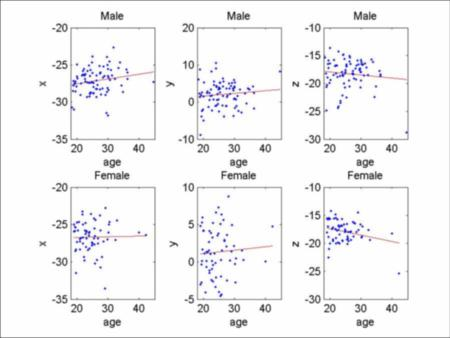Looks like the quadratic effect is caused by one male and one female (ages 45 and 42, respectively) with very low z coordinate.

### VBM data

Here's an example of voxel based morphometry (VBM) data, analyzed in Worsley et al. (2004). There are two groups of subjects: a group of 19 subjects with non-missile trauma, and a group of 19 age and gender matched controls. Damage due to the trauma is expected in white matter, so the data consists of white matter density smoothed 10mm. Naturally we are interested in the difference between trauma and control groups, so the model is particularly simple. First we need a list of the 38 file names: filenames = SurfStatListDir( 'C:/keith/fMRI/francesco/wm2/' ); Since the data is quite big (91 × 109 × 91 × 38 × 4 = 131Mb) we have to be careful about reading the data. The first thing might be to look at just one slice of the data, say the slice with `z=0`, laid out in two groups of trauma and control. To do this, we first read in just the one slice with `z` coordinate `0` from all data sets, which easily fits into memory: [ Y0, vol0 ] = SurfStatReadVol( filenames, [], { [], [], 0 } ); The second parameter, left empty for the moment, is to allow for a mask, while the third specifies that we want all `x` slices, all `y` slices, but just the one slice with `z=0`. We then use `SurfStatViews` (the `s` is for slice) with a last parameter, `layout`, which is a matrix which lays out the slices (in the order in filenames) where you want them in the array of images (0 leaves out an image). The variable `control` indicates with a 1 which files are in the control group, in the order in `filenames`: control=[0 0 1 1 1 1 0 1 1 0 0 0 0 1 0 0 0 1 ... 0 1 0 1 0 1 1 0 1 1 1 0 1 1 1 0 0 0 0 1]; layout = reshape( [ find(1-control) 0 find(control) 0], 5, 8 ) 1 12 19 34 3 9 24 31 2 13 21 35 4 14 25 32 7 15 23 36 5 18 27 33 10 16 26 37 6 20 28 38 11 17 30 0 8 22 29 0 clf; SurfStatViews( Y0, vol0, 0, layout ); title('WM demsity for 19 trauma subjects (left) and 19 controls (right)');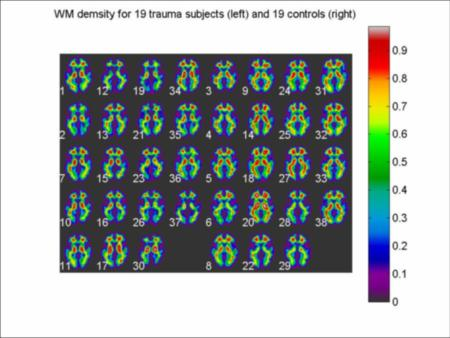Even to the naked eye, it looks like the trauma group has less WM than the control group. To save storage, we now find a mask based on the average white matter density in the control group:

[ wmav, volwmav ] = SurfStatAvVol( filenames( find(control) ) ); `SurfStatView1` is a viewer for both volumetric and surface data with only one view (hence the name). By default it will show three orthogonal slices through the middle of the data: clf; SurfStatView1( wmav, volwmav );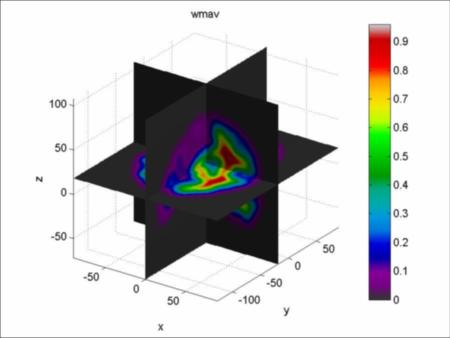Now we need to choose a suitable threshold to mask the white matter. 0.05, i.e. 5% or more white matter, looks like a good choice. To check this, add a thresholded image at 0.05 by leaving out the `clf` command (which clears the figure window):

SurfStatView1( wmav, volwmav, 'datathresh', 0.05 );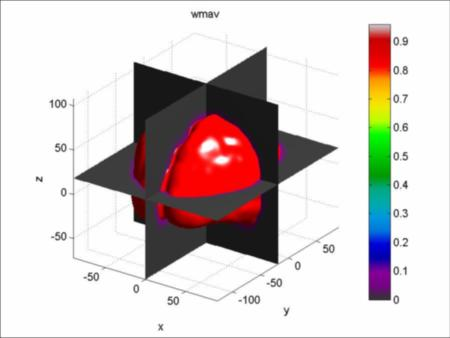Other properties such as the colour and transparency can be modified, see the help of `SurfStatView1`, and strung together in pairs of arguments after `volwmav`.

Now we are ready to read in all the data inside the mask. If there is a lot of data, `SurfStatReadVol` uses memory mapping rather than loading all the data into memory.

[ Y, vol ] = SurfStatReadVol( filenames, wmav > 0.05 ); However by specifying a mask where average WM>0.05, we have cut down storage from 131Mb to 26Mb, so memory mapping is not necessary. If you wanted to speed things up by cutting the storage down even more, you can read in every 2nd voxel using: [ Y, vol ] = SurfStatReadVol( filenames, wmav > 0.05, 2 ); We'll stick with the full data, which gives nicer pictures. Setting up the group factor is easy: Group = term( var2fac( control, { 'trauma'; 'control' } ) ); Now we fit the model and test for a difference between trauma and control. Naturally we want control minus trauma, since we expect WM density to have decreased in the trauma group: slm = SurfStatLinMod( Y, Group, vol ); slm = SurfStatT( slm, Group.control - Group.trauma ); clf; SurfStatView1( slm.t, vol ); title( 'T-statistic, 36 df' );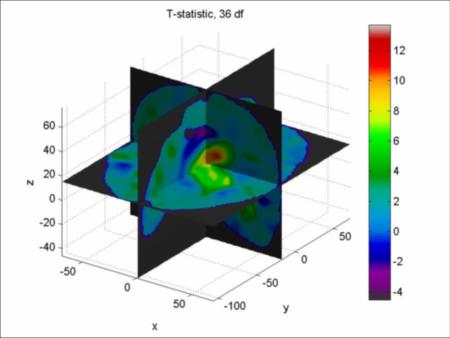Of course you may prefer to write out the T-statistic so you can load it into your own favourite viewer:

SurfStatWriteVol( 'c:/keith/surfstat/data/tstat.mnc', slm.t, vol ); Now for the P-values: clf; SurfStatView1( SurfStatP( slm ), vol ); title( 'P-value<0.05' ); The red blobs are local maxima with P<0.05, and the transparent blue blobs are the clusters with extents that have P<0.05:

The damage is quite extensive, indicating that the white matter surrounding the ventricles has atrophied. To see how much atrophy, let's look at the estimated effect (control minus trauma) and its standard error:

clf; SurfStatView1( slm.ef, vol ); title( 'Control - trauma wm' ); clf; SurfStatView1( slm.sd, vol ); title( 'Control - trauma wm sd (34 df)' );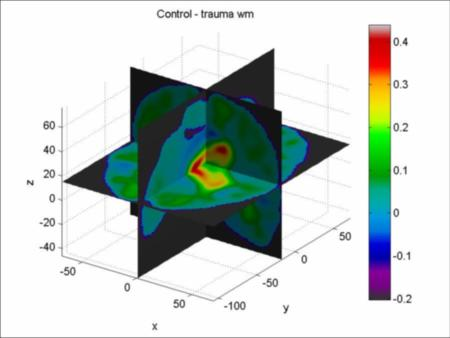You may prefer the more traditional view of slices, say every 10mm from -30mm to 70mm:

layout = reshape( [1:11 0], 3, 4); clf; SurfStatViews( slm.ef, vol, [-30:10:70], layout ); title( 'Control - trauma wm' ); clf; SurfStatViews( slm.sd, vol, [-30:10:70], layout ); title( 'Control - trauma wm sd (34 df)' );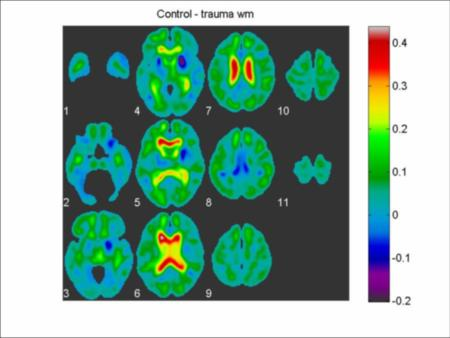It looks like the trauma subjects have lost between 20% and 40% WM, ± ~5%, in the regions surrounding the ventricles.

### DBM data

Here's an example of deformation based morphometry (DBM) data, analyzed in Worsley et al. (2004). This is trivariate volumetric data, where the three components are the deformations required to warp each subject to an atlas standard. There are two groups of subjects: a group of 17 subjects with non-missile trauma, and a group of 19 age and gender matched controls. Unfortunately the subjects in this example are not quite the same as those in the above VBM example. Naturally we are interested in the difference between trauma and control groups, so the model is particularly simple. First a look at the data, which comes in three separate files for the `x`, `y` and `z` deformation components for each subject. We first read in the file names then rearrange them into 17 × 3 and 19 × 3 cell arrays of file names as follows: filenamestrauma = SurfStatListDir( 'C:/keith/fMRI/francesco/DXYZ_TRAUMA/' ); filenamestrauma = reshape( filenamestrauma, 3, 17 )'; filenamescontrol = SurfStatListDir( 'C:/keith/fMRI/francesco/DXYZ_CONTROLLO/' ); filenamescontrol = reshape( filenamescontrol, 3, 19 )'; Let's take a look at slice `z=0` of all the data, arranged in rows of `x`, `y` and `z` deformations for the trauma group, followed by `x`, `y` and `z` deformations for the control group: [ Y0, vol0 ] = SurfStatReadVol( [ filenamestrauma; filenamescontrol ], [], { [], [], 0 } ); layout = [ [ reshape(1:51,17,3); zeros(2,3) ] zeros(19,1) reshape(52:108,19,3) ]' clf; SurfStatViews( Y0, vol0, 0, layout ); title('Rows are x,y,z deformations for trauma (top) and control (bottom) groups');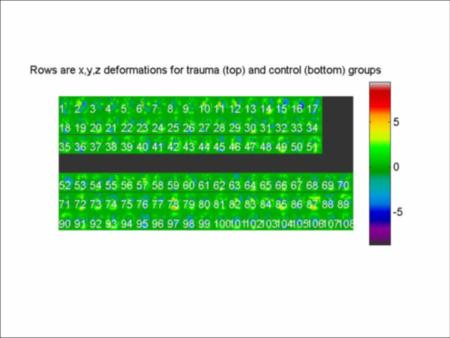It's hard to see any difference in deformations between trauma and control, but at least the data look OK. Next we will need a mask. Since the trauma is expected to atrophy the white matter, let's form a mask by thresholding average white matter density. A file for this has already been created:

[ wm, volwm ] = SurfStatReadVol( 'c:/keith/fMRI/francesco/CNT_AVG_wm_mask.mnc' ); clf; SurfStatView1( wm, volwm );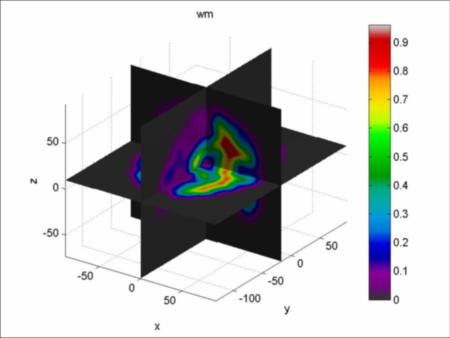0.05, i.e. 5% or more white matter, looks like a reasonable threshold:

SurfStatView1( wm, volwm, 'datathresh', 0.05 );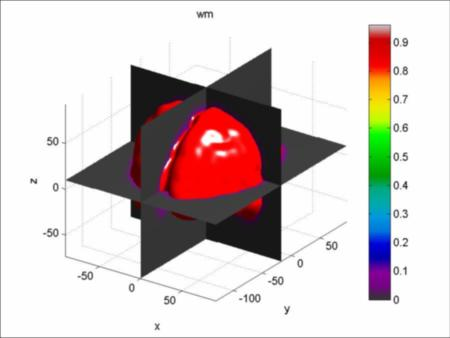Now let's read in all the data, which just exceeds 64Mb, so it is memory mapped:

[ Y, vol ] = SurfStatReadVol( [ filenamestrauma; filenamescontrol ], wm > 0.05 ); Filename: 'C:\keith\fMRI\francesco\DXYZ_TRAUMA\SurfStat\tpb11de523_b7d0_49a1_91ec_0fc7ad1a95c9' Writable: true Offset: 0 Format: {'single' [163814 3 36] 'Data'} Repeat: Inf Data: 1x1 struct array with fields: Data Setting up the group factor is easy: Group = term( var2fac( [ zeros(1,17) ones(1,19) ], { 'trauma'; 'control' } ) ); Now we fit the model and test for a difference between trauma and control: slm = SurfStatLinMod( Y, Group, vol ); slm = SurfStatT( slm, Group.trauma - Group.control ); clf; SurfStatView1( slm.t, vol ); title( 'Hotelling''s T-statistic, 3,34 df' );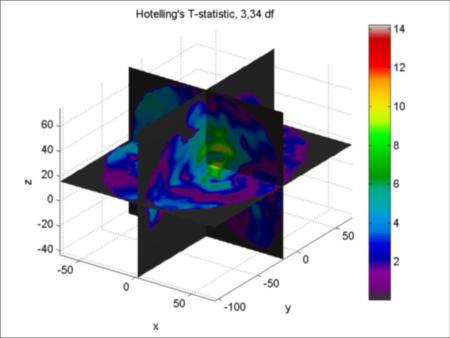Now for the P-values:

clf; SurfStatView1( SurfStatP( slm ), vol ); title( 'P-value<0.05' );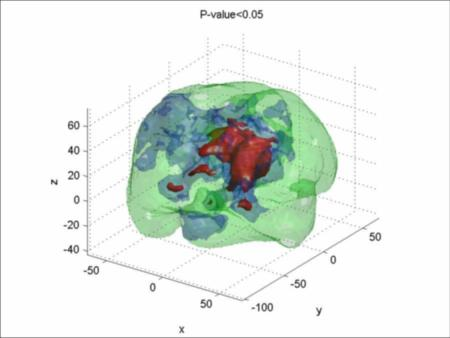The damage is quite extensive, but what are the actual deformation differences? We can visualize the deformations by adding them as little arrows to the image. To do this, let's first select some positions for the arrows. I've chosen positions on a lattice of 10mm intervals using Matlab's `ndgrid`:

[ x, y, z ] = ndgrid( -40:10:40, -50:10:30, -20:10:60 ); Then we get the id's of the nearest point in the image: id = SurfStatCoord2Ind( [ x(:) y(:) z(:) ], vol ); id's outside the mask are set to zero, so to eliminate these: id = id( id>0 ); Now get the actual coordinates of the remaining points: coord = SurfStatInd2Coord( id, vol ); The deformation differences are in `slm.ef` but since the deformations are measured from subject to atlas, i.e. atlas minus subject, we should flip the sign so that we have trauma minus control deformations. Then we can use Matlab's `quiver3` to add the arrows: hold on; quiver3( coord( 1, : ), coord( 2, : ), coord( 3, : ), ... -slm.ef( 1, id ), -slm.ef( 2, id ), -slm.ef( 3, id ), 0, ... 'LineWidth',2, 'Color','yellow' ); hold off;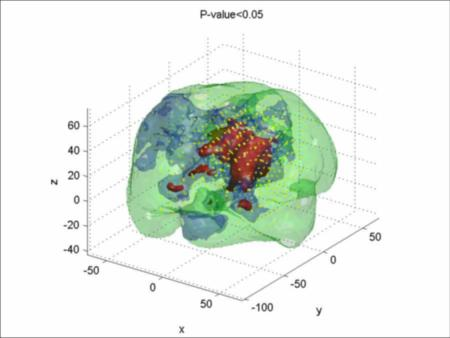The "0" as the 7th argument of `quiver3` ensures that the arrows are drawn to scale, that is, they are the real movements in anatomy, in mm. Let's zoom in by hitting the "+" magnifying glass in the figure tool bar:

It looks like the arrows are pointing out from the centre, indicating that the ventricles of the trauma subjects have expanded, or equivalently, that the white matter surrounding the ventricles has atrophied.

This illustrates nicely how DBM can not only detect where the anatomy has changed, but it can also show how the anatomy has moved, i.e. the direction of the changes.

### PET data

We use SurfStat to analyze the some non-kinetic PET CBF data from Vafee, M.S. & Gjedde, A. (2004). Spatially dissociated flow-metabolism coupling in brain activation. NeuroImage, 21:507-515. This same data has previously analyzed by FmriStat - see here. In this study there were 14 subjects with 5 scans per subject. During the first 4 scans the subjects were given a right-hand finger tapping task at four different frequencies: 1Hz, 2Hz, 3Hz and 4Hz (labeled `h1`, `h2`, `h3` and `h4`). These are to be compared to the 5th scan of rest (labeled `hb`). We can get a list of all the 70 PET data files as follows: filenames = SurfStatListDir( 'C:/keith/fMRI/manou/cbf_non_kin/' ); Since the data is quite big (70 × 128 × 128 × 80 × 4 = 350Mb) we have to be careful about reading the data. The first thing might be to look at just one slice of the data, say the slice with `z=0`, laid out in a task × subjects matrix. To do this, we first read in just the one slice with `z` coordinate `0` from all data sets, which easily fits into memory: [ Y0, vol0 ] = SurfStatReadVol( filenames, [], { [], [], 0 } ); The second parameter, left empty for the moment, is to allow for a mask, while the third specifies that we want all `x` slices, all `y` slices, but just the one slice with `z=0`. We then use `SurfStatViews` with a last parameter, `layout`, which is a matrix which lays out the slices (in the order in filenames) where you want them in the array of images: layout = reshape( 1:70, 5, 14 ) 1 6 11 16 21 26 31 36 41 46 51 56 61 66 2 7 12 17 22 27 32 37 42 47 52 57 62 67 3 8 13 18 23 28 33 38 43 48 53 58 63 68 4 9 14 19 24 29 34 39 44 49 54 59 64 69 5 10 15 20 25 30 35 40 45 50 55 60 65 70 clf; SurfStatViews( Y0, vol0, 0, layout );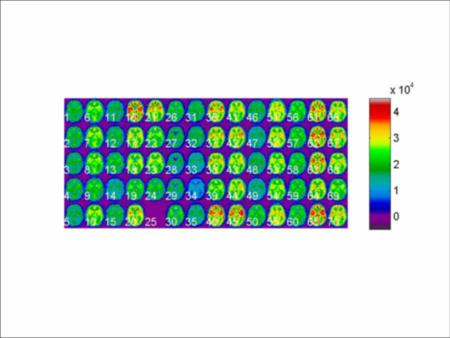It is obvious that there is a bad scan (#25). In the FmriStat analysis, the entire subject subject #5 was dropped from the analysis. Here we shall retain all the data on subject #5, except the bad scan, and do a mixed effects analysis to recover as much information as possible from the data. To exclude the bad scan, #25:

filenames = filenames( [1:24, 26:70] ); To save storage, we first find a mask where all subjects have some data, and only read in this data. To do this we find the minimum across subjects: [ mindata, volmin ] = SurfStatAvVol( filenames, @min, 0 ); The second argument of `SurfStatAvVol` tells the function that we want the minimum over scans (other possible choices are `@max` for the maximum, or `@plus` for the average, which is the default). The third argument replaces NaN ("not a number") by 0. `SurfStatView1` is a viewer for both volumetric and surface data with only one view (hence the name). By default it will show three orthogonal slices through the middle of the data:

clf; SurfStatView1( mindata, volmin );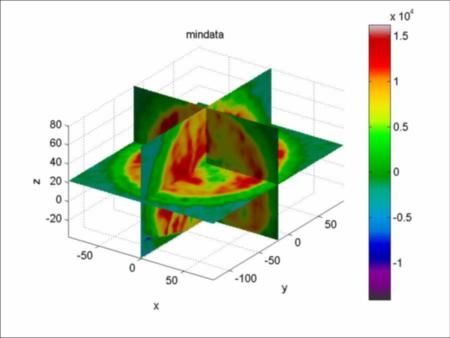Now we need to choose a suitable threshold to mask the interior of the brain. 5000 looks like a good choice. To check this, reset the colour limits as follows:

set( gca, 'CLim', [ 5000 15000 ] );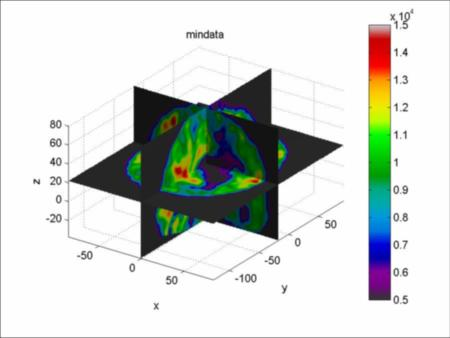Now we are ready to read in all the data inside the mask. If there is a lot of data, as in this case, `SurfStatReadVol` uses memory mapping rather than loading all the data into memory:

[ Y, vol ] = SurfStatReadVol( filenames, mindata>=5000 ); Filename: 'C:\keith\fMRI\manou\cbf_non_kin\SurfStat\tp7e6b2b61_2069_4960_a922_0fac58f6eacc' Writable: true Offset: 0 Format: {'single' [498412 69] 'Data'} Repeat: Inf Data: 1x1 struct array with fields: Data If you had your own mask (1=in, 0=out) in 'mymaskfile', you must read it in as a volume with `SurfStatReadVol1`, extract the data part, then convert to a logical: mask = SurfStatReadVol1( 'mymaskfile' ); [ Y, vol ] = SurfStatReadVol( filenames, logical(mask.data) ); If you want to speed things up, you can read in every 2nd voxel, which doesn't need memory mapping, using: [ Y, vol ] = SurfStatReadVol( filenames, mindata>=5000, 2 ); This would allow you to quickly explore a variety of models. Proceeding with the full data, let's check the slice with `z=0` (0 in `layout`leaves a gap in the array for the deleted scan): layout = reshape( [1:24, 0, 25:69], 5, 14 ); clf; SurfStatViews( Y, vol, 0, layout );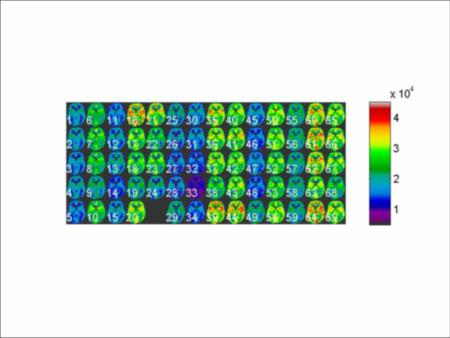It is immediately obvious that the data need normalizing so that all images have roughly the same average intensity, as follows:

Y = SurfStatNorm( Y, [], 'divide'); clf; SurfStatViews( Y, vol, 0, layout );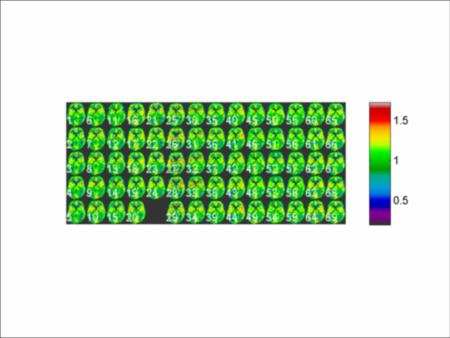Since the design is almost balanced, the simplest way for generating the levels of the factors for subject and condition is to generate them for a balanced design, then delete the levels for the bad scan:

subj = gl( 'Subj', 14, 5, 70); subj = subj( [1:24, 26:70] ) 'Subj1' 'Subj1' 'Subj1' 'Subj1' 'Subj1' 'Subj2' 'Subj2' ... 'Subj13' 'Subj13' 'Subj14' 'Subj14' 'Subj14' 'Subj14' 'Subj14' cond = gl( {'h1','h2','h3','h4','hb'} ,1, 70 ); cond = cond( [1:24, 26:70] ) 'h1' 'h2' 'h3' 'h4' 'hb' 'h1' 'h2' ... 'h4' 'hb' 'h1' 'h2' 'h3' 'h4' 'hb' Let's look at a model with condition as a fixed effect and subject as a random effect: Cond = term( cond ); Subj = term( subj ); M = 1 + Cond + random( Subj ) + I; image( M );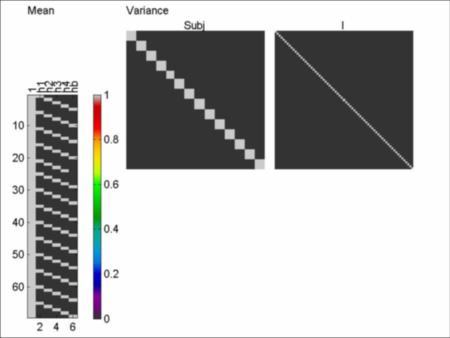Fitting the model, the within-subject correlation is quite high, averaging 0.59:

slm = SurfStatLinMod( Y, M, vol ); clf; SurfStatView1( slm.r, vol ); title('Within-subject correlation') mean( slm.r ) 0.5892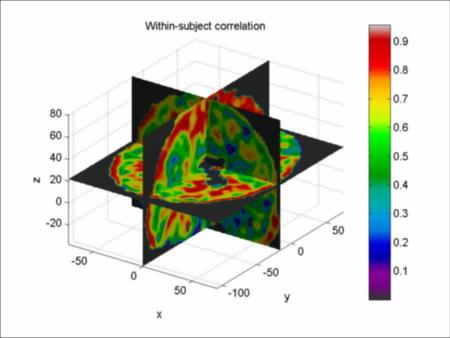Now we test for the difference between the 3Hz condition and the baseline, picking two slices at `z=[-20, 60]` for display:

slm = SurfStatT( slm, Cond.h3 - Cond.hb ); clf; SurfStatView1( slm.t, vol, 'z', [-20 60] ); title('T-statistic for 3Hz - baseline');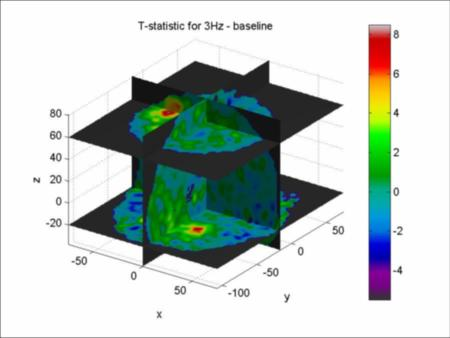To get a better look, let's threshold the T-statistic at 3 and add it to the figure by leaving off the `clf` command (which clears the figure window):

SurfStatView1( slm.t, vol, 'datathresh', 3 ); title('T-statistic for 3Hz - baseline');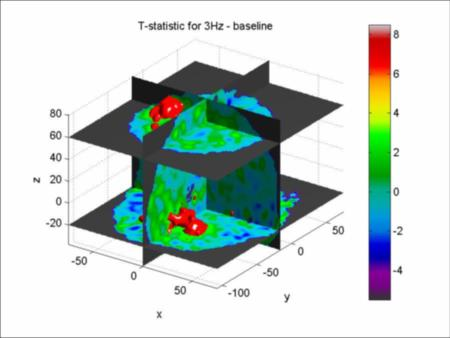SurfStatView1( slm.t, vol, 'mask', 1 ); title('T-statistic for 3Hz - baseline');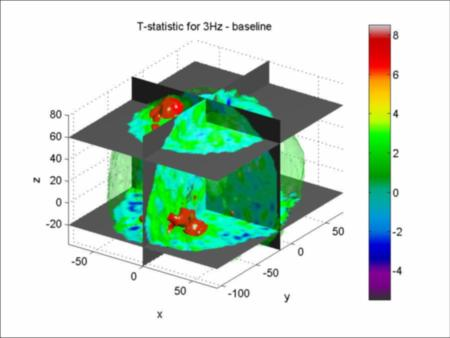Other properties such as the colour and transparency can be modified, see the help of `SurfStatView1`, and strung together in pairs of arguments after `vol`. You may like the more traditional view of slices, say every 10mm:

layout = reshape( [1:11 0], 3, 4); clf; SurfStatViews( slm.t, vol, [-30:10:70], layout );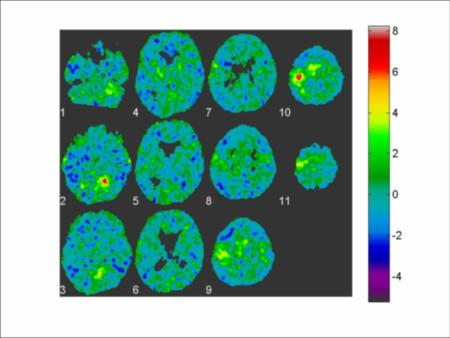Of course you may prefer to write out the T-statistic so you can load it into your own favourite viewer:

SurfStatWriteVol( 'c:/keith/surfstat/data/tstat.mnc', slm.t, vol ); Now we come to the inference. Since the T-statistic comes from a linear mixed effects model, its effective degrees of freedom varies spatially, averaging at 51.2: clf; SurfStatView1( slm.dfs, vol ); mean( slm.dfs ) 51.1516 title('Degrees of freedom, mean = 51.2');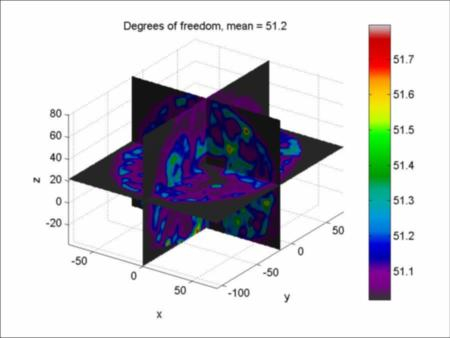Note that the mean effective df is slightly higher than the fixed effects df, which would be 69 - 14 - 5 + 1 = 51. This is because the mixed effects model has recovered some of the between-subject variation to increase the amount of information about the contrast. Finally the P-values and Q-values are:

[ pval, peak, clus] = SurfStatP( slm ); term( clus ) clusid nverts resels P ------------------------------------------ 1 2137 13.1781 4.3378e-006 2 1321 10.5468 4.7328e-006 3 731 4.50147 0.00021312 4 108 0.930881 0.18245 5 62 0.658461 0.39604 ... term( peak ) + term( SurfStatInd2Coord( peak.vertid, vol )', {'x','y','z'}) t vertid clusid P x y z -------------------------------------------------------------------- 8.5028 454759 1 5.8716e-006 -34.84 -19.44 58.5 7.5756 444601 1 0.000124403 -36.18 -17.72 55.5 7.4453 64818 2 0.000189549 16.08 -53.84 -19.5 7.3037 467879 1 0.000299923 -33.5 -26.32 63 7.2892 463681 1 0.000313491 -34.84 -26.32 61.5 6.461 72281 2 0.0044887 20.1 -55.56 -18 6.3465 64638 2 0.00644978 21.44 -57.28 -19.5 6.1895 475829 1 0.0106013 -28.14 -16 66 5.9699 438053 1 0.0210979 -34.84 -38.36 54 5.6383 445139 3 0.0587249 -4.02 -7.4 55.5 5.4934 121064 2 0.0911926 6.7 -62.44 -9 ... clf; SurfStatView1( pval, vol ); title('P-value < 0.05'); The red blobs are local maxima with P<0.05, and the three transparent blue blobs are the three clusters with extents that have P<0.05:

clf; SurfStatView1( SurfStatQ( slm ), vol ); title('Q-value < 0.05');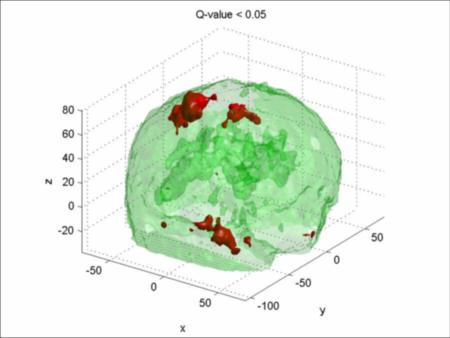Finally, just for amusement, let's add a surface to the last image:

avsurf = SurfStatReadSurf( 'c:/keith/fmri/icbm/av.obj' ); load c:/keith/surfstat/data/meanthick SurfStatView1( meanthick, avsurf );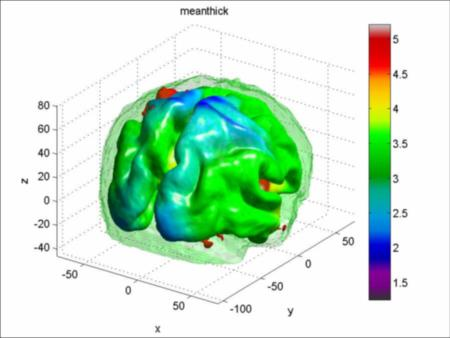### PET data: more elaborate linear models

In the above analysis, we only compared the scan at 3Hz with baseline. We might be interested in a linear effect of frequency. Since we are exploring the data, it is better to read in every second voxel, which avoids memory mapping and speeds up our analysis: [ Y, vol ] = SurfStatReadVol( filenames, mindata>=5000, 2 ); There are two ways of doing this, as discussed in a similar analysis of fMRI data. The first is to simply look at a linear contrast in the four frequencies, as follows: slm = SurfStatLinMod( Y, M, vol ); slm = SurfStatT( slm, -3*Cond.h1 - Cond.h2 + Cond.h3 + 3*Cond.h4 ); mean( slm.dfs ) 51.0016 clf; SurfStatView1( slm.t, vol, 'z', [-20 60] ); title('T-statistic for a linear contrast in Hz, 51.0 df');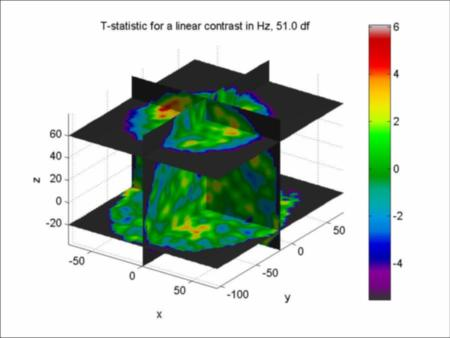An alternative is to make up a new linear model with an explicit linear variable for frequency. To do this, we extract the frequencies from the `cond` variable, and set the baseline to 0:

hz = fac2var( cond ); hz( hz==5 ) = 0; Hz = term( hz ) hz ---- 1 2 3 4 0 1 2 3 4 0 1 2 ... This will be our frequency variable. Now we create a factor for the baseline, so that we can "model out" the baseline scan by giving it a separate coefficient: Baseline = term( var2fac( hz==0, {'h1234', 'hb'} ) ) h1234 hb ----------- 1 0 1 0 1 0 1 0 0 1 1 0 1 0 1 0 1 0 0 1 1 0 1 0 ... The linear model with `Baseline + Hz` does not force the intercept of the Hz effect to go through the baseline data, since `Hz=0` might not produce the same response as the baseline. In other words, the intercept of the Hz effect is modeled separately from the baseline: M1 = Baseline + Hz + random( Subj ) + I; clf; image( M1 );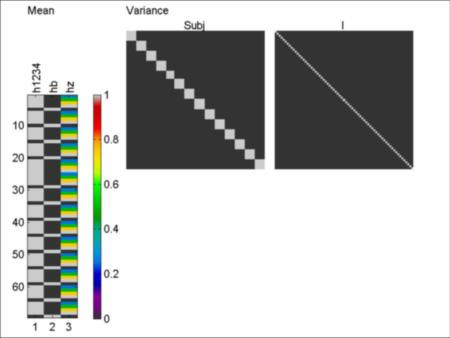Now we fit the model in the usual way:

slm1 = SurfStatLinMod( Y, M1, vol ); slm1 = SurfStatT( slm1, hz ); mean(slm1.dfs) 53.0016 clf; SurfStatView1( slm1.t, vol, 'z', [-20 60] ); title('T-statistic for a linear effect in Hz, 53.0 df');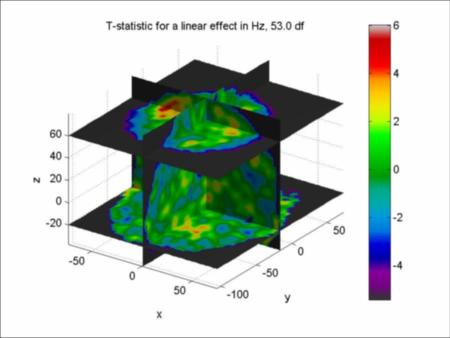As we can see, the two T-statistics, the first a linear contrast, the second a linear effect of Hz, are almost identical. The main difference is that the df of the first is lower (51) than the second (53). Why? The reason is that the first model has a separate effect for each level of Hz, in other words, it allows for an arbitrary non-linear effect of Hz; the second only has a linear effect of Hz, so it has ~2 more df for the error. Which is better? As usual, it's a trade-off; the first will have an sd that is less biased, but less accurate; the second will have an sd that might be biased (if the the true Hz effect is non-linear, e.g. quadratic) but more accurate. Luckily both methods give almost identical results. Here are the P-values for the second method:

[ pval, peak, clus ] = SurfStatP( slm1 ); term( clus ) clusid nverts resels P ---------------------------------------- 1 185 7.3794 8.7721e-006 2 106 1.5688 0.027234 3 39 1.3454 0.047272 4 46 1.3417 0.047724 5 25 1.3279 0.049443 6 19 1.0097 0.11653 7 18 0.75241 0.24668 ... term( peak ) + term( SurfStatInd2Coord( peak.vertid, vol )', {'x','y','z'}) t vertid clusid P x y z ----------------------------------------------------------- 6.0356 14055 38 0.00497395 -69.68 -46.96 -10.5 5.9801 27761 2 0.00609785 -61.64 21.84 7.5 5.4596 56971 1 0.0404088 -40.2 -19.44 58.5 5.4004 58126 1 0.0499989 -37.52 -19.44 61.5 5.3777 21434 6 0.0541458 26.8 14.96 -1.5 5.1428 55731 1 0.125335 -42.88 -16 55.5 ... figure(2); clf; SurfStatView1( pval, vol ); title('P-value < 0.05');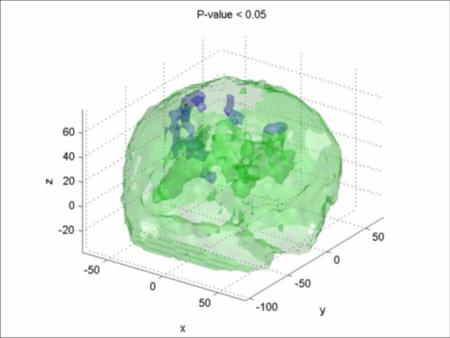The activated local maxima are almost too small to see. Let's plot the data at the maximum T-statistic:

clf; SurfStatPlot( hz, Y( :, 14055 ), M1 ); ylim([0.3 0.9]);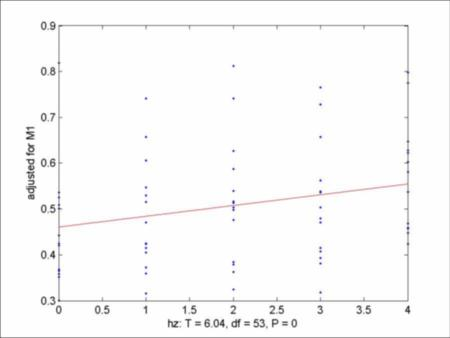Although the T-statistic is high (6.04), the data doesn't seem to be highly correlated. This is because the Y values are adjusted only for the fixed effects, not the fixed and random (subject) effects. One way to adjust Y for subject is to treat subject as fixed:

MFixed = Baseline + Subj + I; clf; SurfStatPlot( hz, Y( :, 14055 ), MFixed ); ylim([0.3 0.9]);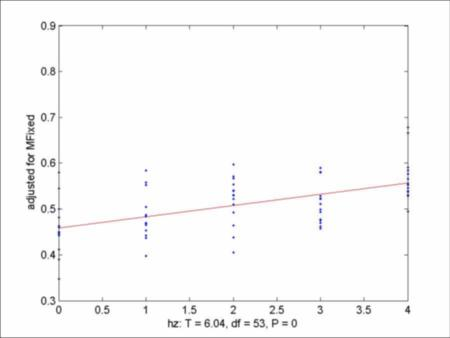The command `ylim` sets the same y limits, so we can compare the plots. The slopes are almost identical, but now the data in the second plot are far less variable because the subject effect has been removed, so now the data looks more correlated.

Let's look at a quadratic effect of Hz. Again there are two ways of doing it, either through contrasts or through an explicit quadratic model. Here's the first approach, through a quadratic contrast:

slm = SurfStatT( slm, Cond.h1 - Cond.h2 - Cond.h3 + Cond.h4 ); mean( slm.dfs ) 51.0016 clf; SurfStatView1( slm.t, vol, 'z', [-20 60] ); title('T-statistic for a quadratic contrast in Hz, 51.0 df');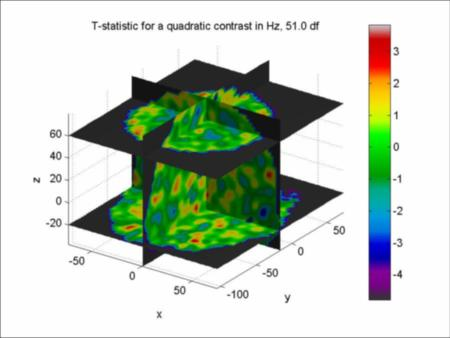Here's the model for the second approach, though a quadratic model:

M2 = Baseline + Hz + Hz^2 + random( Subj ) + I; clf; image( M2 );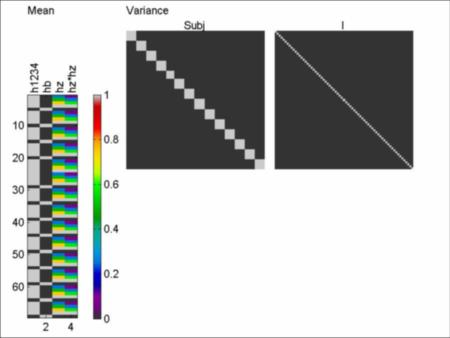Here's the analysis of the quadratic effect:

slm2 = SurfStatLinMod( Y, M2, vol ); slm2 = SurfStatT( slm2, hz.^2 ); mean(slm2.dfs) 52.0016 clf; SurfStatView1( slm2.t, vol, 'z', [-20 60] ); title('T-statistic for an effect of Hz^2, 52.0 df');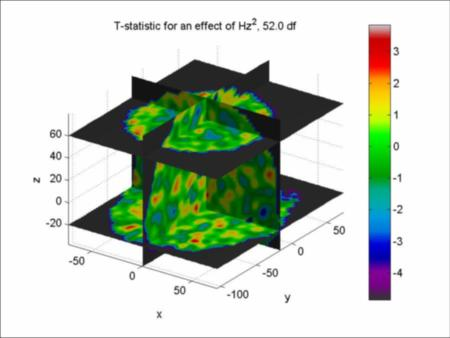The analyses are almost identical, apart from the different df, an neither shows any significant quadratic effect.

Finally, let's look at a cubic effect of Hz, done both ways:

slm = SurfStatT( slm, -Cond.h1 + 3*Cond.h2 - 3*Cond.h3 + Cond.h4 ); mean( slm.dfs ) 51.0016 M3 = Baseline + Hz + Hz^2 + Hz^3 + random( Subj ) + I; slm3 = SurfStatT( SurfStatLinMod( Y, M3, vol ), hz.^3 ); mean(slm3.dfs) 51.0016 Why are the mean dfs the same? In fact the fits of the models, `slm.SSE`'s, are the same as well. The answer is that they are exactly the same model! A cubic model in Hz (`M3`) is identical to a separate mean for each of the four levels of Hz (`M`). The only difference is that the models are parameterized in different ways. But the fitted models are identical. Why? A cubic can be fitted exactly to any data at four points.

### Future features

• Support for Gifti file format.
• F tests for mixed effects models.
• Multivariate mixed effects models.
• I would really appreciate any feed-back!

### References for random field theory

• Adler, R.J. and Taylor, J.E. (2007). Random fields and geometry. Springer.

• Adler, R.J., Taylor, J.E. and Worsley, K.J. (2008). Random fields, geometry, and their applications. In preparation.

• Hagler, D.J., Saygin, A.P. and Sereno, M.I. (2006). Smoothing and cluster thresholding for cortical surface-based group analysis of fMRI data. NeuroImage, 33:1093-1103.

• Hayasaka, S., Phan, K.L., Liberzon, I., Worsley, K.J. and Nichols, T.E. (2004). Non-Stationary cluster size inference with random field and permutation methods. NeuroImage, 22:676-687.

• Taylor, J.E. and Adler, R.J. (2003), Euler characteristics for Gaussian fields on manifolds. Annals of Probability, 31:533-563.

• Taylor, J.E. and Worsley, K.J. (2007). Detecting sparse signal in random fields, with an application to brain mapping. Journal of the American Statistical Association, 102:913-928.

• Worsley, K.J., Andermann, M., Koulis, T., MacDonald, D., and Evans, A.C. (1999). Detecting changes in non-isotropic images. Human Brain Mapping, 8:98-101.